• 非线性可视化（5）非线性系统分岔图
千次阅读
2021-11-07 00:52:09在前面 非线性可视化（3）混沌系统 这一篇文章中，介绍了一个系统因为某个常数的改变，从而导致整个系统发生变化的例子。比如Duffing系统，随着阻尼d的增大，系统由混沌变为倍周期，又变为周期运动。想要描述系统的某个参数变化，导致的系统本质的改变，就需要引入分岔图

• 1 离散系统的分岔图

离散系统中的混沌现象非常普遍，通常经过简单的非线性方程，然后进行反复迭代就很容易出现。这种迭代产生的混沌十分简单，甚至不需要编程，随手用excel就可以展示其混沌现象：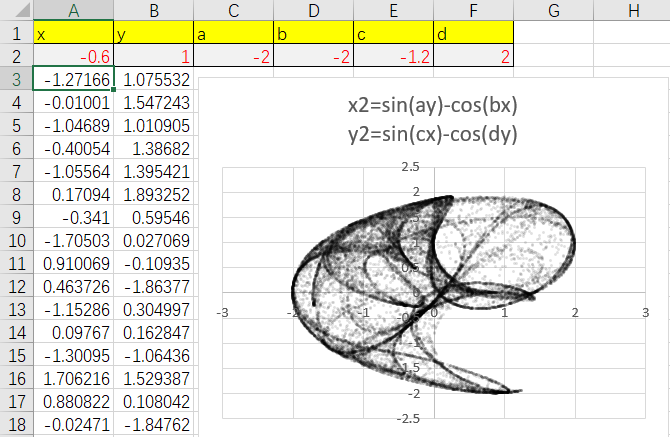离散系统的分岔图绘制方法，就是记录不同参数改变后，系统稳定点的位置。然后绘制出散点图，其中横坐标为参数，纵坐标为每个参数对应的稳定位置。

稳定位置常见计算方法有两种。举个例子来说明，一种是先分布100个不同的种子点，迭代999次，然后记录这100个点现在的位置。另一种是只有1个种子点，迭代999次，然后记录这个点999次里每一次的位置。

首先以一个一维离散系统为例，采用经典的Logistic系统，迭代方程如下：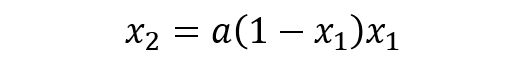方程的参数为a。对于每一个a，都先在[0,1]区间内生成若干xi，然后经过长时间迭代直至收敛后，记录下每一个xi的值。效果如下：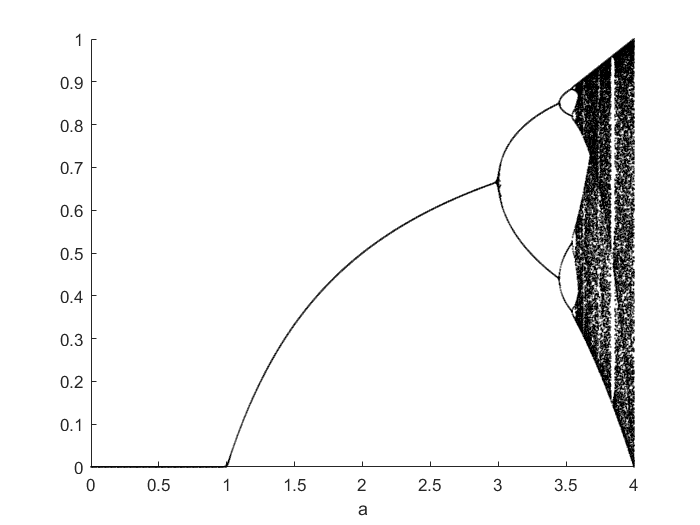一维的分岔图非常的直观，因为变量只有1个。对于二维的分岔图，需要先将结果投影到一维上，然后再绘制。

下面举一个二维离散系统的例子，用的是Henon系统为例，迭代方程如下：这里固定b=0.3，来改变a的值。这里由于是二维，采用第一种方法，每个维度都铺上初始点的话，计算量会比较大。所以采用第二种方法，用单个初始值，经过迭代后记录每一步过程。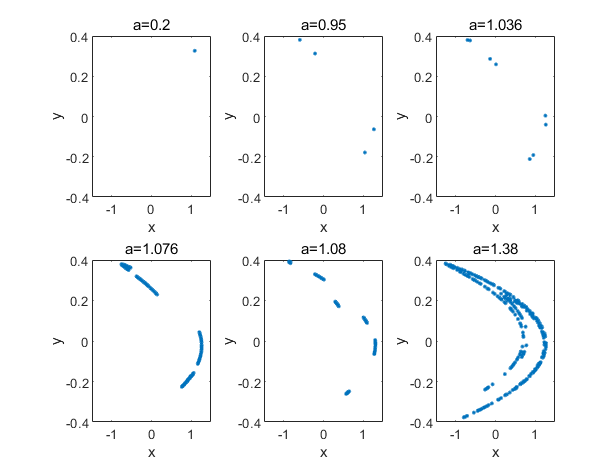上图为选取不同的a值，观察其x、y值随着迭代的变化。当a=0.2时，系统为能够收敛在一个固定的点上。当a=0.95，系统最终在4个不同点上来回跳动。当a=1.036，系统变为在8个点上来回跳动。当a=1.076，系统变为在几根线段上来回跳动。直到a=1.38，系统开始进入混沌。

我们可以只取y值，把这些点投影到1维。然后就可以仿照前面的一维分岔图，绘制出Henon系统的分岔图，完整代码见文末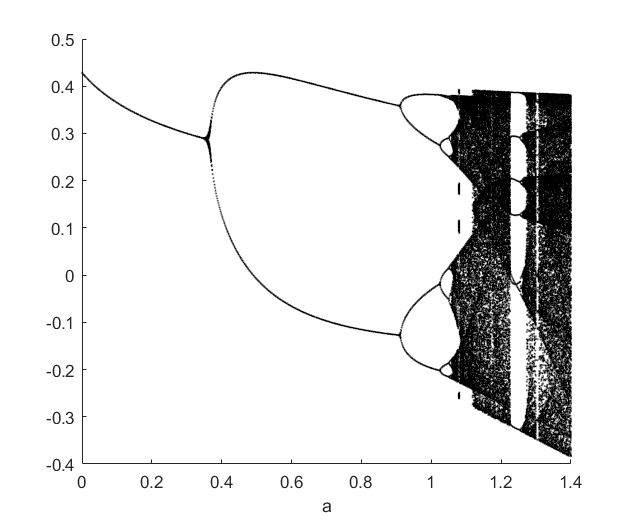• 2 连续系统的分岔图

连续系统的分岔图做法需要参考离散系统分岔图的方法。首先将连续系统降维为离散系统，然后再降维为一维点分布，然后绘图。其中通常参考上面二维离散系统的散点分布图，利用连续系统的庞加莱截面来替代。这也是有些地方说庞加莱截面是沟通连续与离散的桥梁的直观体现。

因此，连续系统的分岔图绘制方法分为三步，首先计算出庞加莱截面，然后投影为一维的点分布，然后绘制到分岔图上。

这里以Rossler方程为例，依然固定a=0.1，b=0.1，然后改变c的值，做系统的分岔图如下，完整代码见文末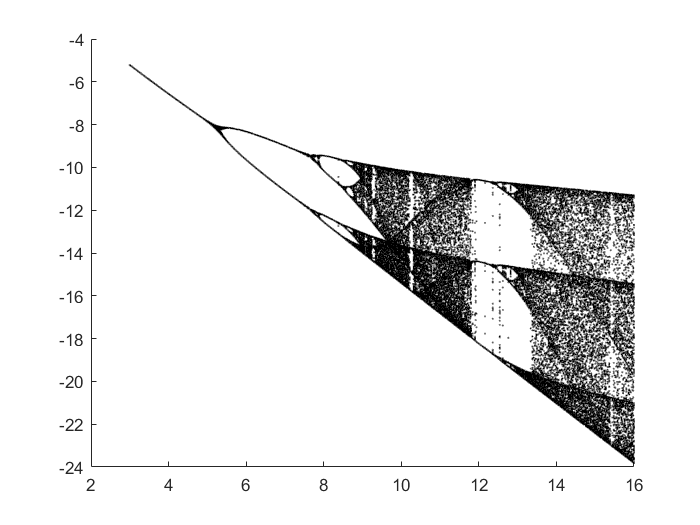一般庞加莱截面的位置选取会改变分岔图的样子，但是通常不会改变分岔点的位置。因为分岔点的位置是由系统本身所决定。

非线性可视化这个专题就先到此为止，还剩下两个非线性分析常用的方法没有介绍：功率谱法和拉雅普诺夫指数法。这两个都不属于可视化的范围内，所以这次没有写到，之后可能有机会再单独写一篇。其中功率谱法一般就是直接一个fft求出频率，然后把幅值平方，观察信号的频谱特性。李雅普诺夫指数法则是一种定量化分析系统分岔的方法，类似于分岔图，但是可以计算出一个数。李雅普诺夫指数反映开始相邻的两个点随着系统迭代后，两个点距离是变大还是缩小。

这个方向可能比较小众，很多地方需要事先建立模型方程。混沌这方面很多方法看似很成熟，但是都不太容易说出个所以然。希望能够帮到涉及到信号振动之类研究的，同时想分析非线性的同学们。

参考资料：

https://blog.csdn.net/xiangger/article/details/113664682

https://wenku.baidu.com/view/0bf3f494172ded630b1cb6b1.html

Santo Banerjee. Applications of Chaos and Nonlinear Dynamics in Engineering[M]. Springer, 2011

Ali.H.Nayfeh. Applied Nonlinear Dynamics Analytical, Computational, and Experimental Methods[M]. 1995.(用到了P284里面的Henon系统)

计算物理基础-第10章第77讲（北京师范大学）（中国大学MOOC）计算物理基础_北京师范大学_中国大学MOOC(慕课) (icourse163.org)

詹姆斯·格雷克. 混沌：开创新科学[M].离散与连续系统分岔图源代码如下：

离散系统的分岔图源代码：python 算法 机器学习 java
更多相关内容
• 非线性动力学幅频、相图、岔常用程序汇总,非线性系统分岔图,matlab源码.zip
• 为解决传统方法在求解非线性振动系统同(异)宿分岔问题过于复杂的问题,以双曲函数摄动法为基础,通过解析非线性振动系统派生方程,再对得出的解析解进行摄动得到最终解析式,提出了计算非线性振动系统同(异)宿轨道解析式...
• 分叉、Lyapunov指数谱和离散时域波形 从混沌稳定性控制的角度,本文研究了采用斜坡补偿的Buck、 Boost和Buck-boost三类基本开关变换器的稳定性,建立了其离散映射选代模型,得到了开关变换器电路的稳定性判据和补偿...
• 理论归根结底是从实践发展而来的,它来之于实践但又反过来指导实践。控制理论的发展又一次说明了这一真理。

## 综述

远在控制理论形成之前,就有蒸汽机的飞轮调速器、鱼雷的航向控制系统、航海罗经的稳定器、放大电路的镇定器等自动化系统和装置。这些都是不自觉地应用了反馈控制概念而构成的自动控制器件和系统的成功的例子。但我们何尝知道在控制理论形成之前的漫长岁月中,由于缺乏理论指导而失败了的无数次的实践和尝试呢?

1920~1940s 20世纪20年代到40年代,马克斯威尔对装有调速器的蒸汽机系统动态特性的分析、马诺斯基对船舶驾驶控制的研究都是控制理论的开拓性工作。奈奎斯特、伯德等人对单回路反馈系统的研究结果显示出反馈控制即使在系统情况知之不多的条件下也可以得到较好的性能。

1940~1950s 维纳对控制理论作出了创造性的贡献。他的控制论概念提供了一个可以把控制问题和通信问题统一考虑的框架。他同时也发展了在有噪声的情况下信号的滤波、预报和平滑的方法,其后又利用了当时刚提出的平稳随机过程,最后建立了信息的伯德一香农概念。

1950~1960s 控制理论发展的转折时期。第二次世界大战后华尔德的序贯分析和贝尔曼的动态规划是转折时期的开端。这些理论受到最优统计决策和资源分配中的序贯规划问题研究的激发。它们在概念上的贡献是考虑了一大类以初始状态参数化了的动态优化问题。这个理论的中心问题是建立最优性能的动态规划方程,从它的解就可以确定最优反馈控制规律。与此同时,优化领域中另一个长期被忽视的强调不等式约束的线性和非线性规划也开始得到发展。这个领域的研究人员首先设计了便于计算机计算的数值方法,这种方法后来在控制中变得十分有用。
苏联学者在20世纪50年代对包含非线性特性、饱和作用和受到限制的控制等因素的系统的最优瞬态的研究表现出很大的兴趣。这些学者的研究讨论导致了庞特里亚金的“极大值原理”。这个原理打开了系统地研究受到状态与控制两方面的约束而使用不连续控制函数的最优轨迹的大道。这些又紧密地和变分法联系,又进一步刺激了与非线性泛函分析相关的更抽象的优化问题的理论的研究。极大值原理的最大贡献可说是20世纪50年代和60年代对于大量轨迹优化数值计算方法的研究的冲力。这种研究最后导致许多空间载运器的成功的设计,其中包括阿波罗计划和宇航飞行计划
显示控制理论转折时期的另一个里程碑是20世纪50年代后期卡尔曼(卡尔曼-一布西)滤波器的发现。早期滤波器设计的维纳理论受到关于平稳随机过程的假设和要求解积分方程或分解傅氏变换的限制。卡尔曼滤波器则不受这些限制,而且可以在小型计算机上当作序贯算法来实现。它的设计在于求解矩阵黎卡提方程。用对偶理论可以得到以同样方程表达的线性反馈控制。这些思想在世界上有巨大影响,它推动了有关反馈控制和滤波的大量研究工作,导致了控制理论的许多实际应用成果。
最近25年线性系统理论的研究非常活跃。自从引人了能控性、能观性、状态实现、线性二次型高斯调节问题的概念之后,这一领域已成为整个控制理论发展的概念基础,而且还成为将成果普遍化到非线性和分布参数系统上去的标准典范和对所有新的控制规范的试验基础。同时,它本身还在继续发展,不断提出新概念、精确的结果和算法。线性系统的几何方法已引出了超不变性、能控性子空间、干扰去耦、非关联控制等重要新概念和对高放大反馈系统的渐近分析方法。与此相关的是线性控制问题的数值分析方面的重要工作。近年来,许多先进线性理论的计算算法已形成商品软件,可以在各种型号计算机(包括个人计算机)使
现在已在非线性常微分方程描述的反馈控制系统的研究中引人了微分几何、李代数、非线性动力学等方法,并得到了很大进展,解决了反馈线性化和非线性去耦问题,也在能控性研究上得到更精确的结果。采用非线性动力学的方法已将反馈镇定作用推广到反馈不能线性化的非线性系统上去。

1960~1970s 将线性二次型理论推广到无穷维系统(即以偏微分方程、泛函微分方程、积分微分方程和在巴拿赫空间的一般微分方程描述的系统)的工作得到很大进展。这一类研究工作是沿着好几条路分别进行的。有人试图得到能为大类无穷维系统应用的一般的算子形式;而另一些人则从一些特殊方程开始做起,如用波动方程或时延微分方程,企图在进行更普遍的形式的研究之前能从具体问题的结构中得到一些启发。经过一段时间的研究已弄清不可能找到一种解求所有无穷维问题的普遍形式,而只能是具体问题具体求解,由此引出了诸如解的常规性、各种无穷维的近似方案的有效性、变分形式等细节研究。目前研究的是以线性偏微分方程或相对简单的迟延方程描述的只能在空间的边界上加以观察和控制的系统。至于对非线性无穷维系统的控制问题的研究,只有在出现了概念上的突破后才谈得到。

偏微分方程的另一方面工作是用包含连续时间和空间变量的动态规划方法推导出来的最优化方程。这一方程也叫哈密尔顿-一雅可比一一贝尔曼方程,已成为先驱分析家的激励的源泉。这些分析家已提出了“粘性解的概念”。如果他们的方法最终能解决哈密尔顿-一雅可比一贝尔曼方程的求解,那么就会有另一种设计非线性反馈控制的工具。

凸分析为控制理论和变分法提供了新方法,也为它们通向数学规划和运筹学的数值分析架起了桥梁。在20世纪70年代早期,凸分析就扩展到“非光滑分析”中去,形成了解决长期末解决的最优控制问题的一个新基础。20世纪60年代发展起来的变分不等式理论在自由边界问题的研究中显示了功效,

1970~1980s 非线性滤波的研究,继续扩展了卡尔曼滤波器,并向它注人了许多新思想。最优控制问题的随机形式在20世纪70年代和80年代吸引了许多学者的兴趣。这一领域是当前最活跃的领域之一。在应用方面,随机控制理论的概念框架已开始对大规模交互关联的动力系统的控制起了影响。

代数在发展更有效的线性控制理论上有多方面的建树。环和模的概念的引人精确地重构了早期获得的有关能控性和能观性的结论。像多项式环上因子分解那样的代数计算方法近来变得很重要。代数几何方法在多变量系统奈查斯特稳定准则和系统辨识中参数化问题的求解方面起了重要作用。

20世纪70年代末80年代初,反馈控制的设计问题经历了一个重新修正的过程。在基于微分方程的状态空间方法普及了多年之后,基于输入输出或频率分析的设计方法又重新抬头。这种方法显得和健壮控制研究有较完善的配合,因为它允许对所有镇定控制器参数化,并可以从中选择其性能在所有频率范围内都一致符合要求的一个控制器。鲁棒控制中的 H ∞ H_{∞} 方法采用了插人理论和复值函数理论(即所谓 H ∞ H_{∞} 空间),其理论深度和实用重要性使此理论成为20世纪80年代重要成果之一。

随着人工智能的发展和引入了新的计算机结构,控制理论和计算机科学的联系愈来愈密切。近来已有一些专家系统可以自动寻求最优随机控制和滤波问题的理论解和数值解。在控制框架上将符号运算和数值运算相结合的研究工作正在开展。智能控制的概念也在发展,其目标之一是将当前的控制理论与尚未成形的人工智能成功地合成一体。离散事件系统理论架起了一座通向扩展了的状态机器理论的桥梁,在将来可能为评价计算机系统的性能提供一个建模工具。

## 分叉图、Lyapunov指数谱和离散时域波形图

分叉图、Lyapunov指数谱和离散时域波形图

从混沌稳定性控制的角度,本文研究了采用斜坡补偿的Buck、 Boost和Buck-boost三类基本开关变换器的稳定性,建立了其离散映射选代模型,得到了开关变换器电路的稳定性判据和补偿斜坡斜率的表达式。基于Matlab,利用分叉图、Lyapunov指数,谱和离散时域波形图,清晰地描绘出引入斜坡补偿后开关变换器电路稳定性能的改变情况。仿真分析与理论研究两者结果完全一致,表明在开关变换器电路中引入补偿斜坡电流(或电压)能有效地控制开关变换器的稳定性,拓宽开关变换器的稳定工作区域,实现了斜坡补偿的稳定性控制

## 离散映射模型和稳定性控制判据

参考：杨汝. “峰值电流控制模式中斜坡补偿电路的设计.” 电力电子技术 35.3 (2001): 35-38.
如果时钟周期与RLC电路的时间常数相比足够小,可以认为电容C的容量足够大,从而在一个开关周期内可以认为电容电压恒定不变,输出部分可以用一个直流电源V。表示。在这种情形下,开关变换器变成一维系统。
对于开关DC-DC变换器电路,可以利用时钟周期同步采样获得离散模型。本文通过一个简单的一维映射来阐述在电流模式控制Buck变换器的
采样数据模型中引入斜坡补偿后的变化。

### 控制系统的状态空间分析与综合

第1章~第7章涉及的内容属于经典控制理论的范時,系统的数学模型是线性定常微分方程和传递函数,主要的分析与综合方法是时域法、根轨迹法和频域法。经典控制理论通常用于单输入-单输出线性定常系统,其缺点是只能反映输人、输出间的外部特性,难以揭示系统内部的结构和运行状态,不能有效处理多输入-多输出系统、非线性系统、时变系统等复杂系统的控制问题。

控制方法特点
经典控制理论经典控制理论通常用于单输入-单输出线性定常系统,其缺点是只能反映输人、输出间的外部特性,难以揭示系统内部的结构和运行状态,不能有效处理多输入-多输出系统、非线性系统、时变系统等复杂系统的控制问题。
状态空间它是以系统内,部状态为基础进行分析与综合的控制理论，最优控制，最优估计与滤波

随着科学技术的发展,对控制系统速度、精度、适应能力的要求越来越高,经典控制理论已不能满足要求。1960年前后,在航天技术和计算机技术的推动下,现代控制理论开始发展,一个重要的标志就是美国学者卡尔曼引人了状态空间的概念。它是以系统内,部状态为基础进行分析与综合的控制理论,其中有两个重要的内容,
(1)最优控制:在给定的限制条件和评价函数下,寻求使系统性能指标最优的控制规律。
(2)最优估计与滤波:在有随机干扰的情况下,根据测量数据对系统的状态进行最,优估计。
本章讨论控制系统的状态空间分析与综合,它是现代控制理论的基础。

### 控制系统的李雅普诺夫稳定性分析

稳定性描述系统受到外界干扰,平衡工作状态被破坏后,系统偏差调节过程的收敛性。它是系统的重要特性,是系统正常工作的必要条件。
经典控制理论用代数判据、奈氏判据、对数频率判据、特征根判据来判断线性定常系统的稳定性,用相平面法来判断二阶非线性系统的稳定性。这些稳定性判据无法满足以多变量、非线性、时变为特征的现代控制系统对稳定性分析的要求。

1892年,俄国学者李雅普诺夫建立了基于状态空间描述的稳定性理论,提出了依赖于线性系统微分方程的解来判断稳定性的第一方法(称为,间接法)和利用经验和技巧来构造李雅普诺夫函数借以判断稳定性的第二方法(称为直,接法)。
李雅普诺夫提出的这一理论是确定系统稳定性的更一般的理论,不仅适用于单变量、线性、定常系统,还适用于多变量、非线性、时变系统。它有效地解决过一些用其他方法未能解决的非线性微分方程的稳定性问题,在现代控制系统的分析与设计中,得到了广泛的应用与发展
早在1892年，俄国有一个叫李雅普诺夫的学者发表了一篇著名的文章《运动稳定性一般》问题，建立了关于运动稳定的一般理论，光看这个文章的名字就不一般，也确实，在尔后百余年，这个理论在数学、力学和控制理论中全面开花，已经成为稳定性研究方向的基础性理论，俄罗斯人对于数学上和工程上的直觉确实令人赞叹。

李雅普诺夫稳定性： 系统的李雅普诺夫稳定性指的是系统在平衡状态下受到扰动时，经过“足够长”的时间以后，系统恢复到平衡状态的能力。

## 平衡点

一个控制系统就和一个社会一样，稳定性是首先要解决的重要问题，是其他一切工作的基础。稳定性问题的字面意思很好理解了，那就是系统在受到扰动后，能否能有能力在平衡态继续工作。大家都知道，历史上社会改革成本很高，且以失败者居多，从控制论的角度来看，就是对社会这个大系统的稳定性研究不够，导致扰动发生后，社会发散了。
稳定性是相对于平衡点而言的，那什么是平衡点呢？我们以发射火箭为例：
火箭的简化模型可以看成是一个倒立摆，如下图所示，在最低端施加控制力，来保持其在竖直方向的角度可控。但是,如果你仔细看,还有一个点,[0 0]'肯定是数学解,但是似乎在图上并没有明显的显示出来,这是什么原因呢?–这代表着火箭竖直放置,且没有扰动,常识告诉我们这是一个极不稳定的点,就像你把铅笔立在桌子上,稍微风吹草动就倒了,而数值求解的时候,几乎寻找不到这个点。

### 李雅普诺夫稳定(1892)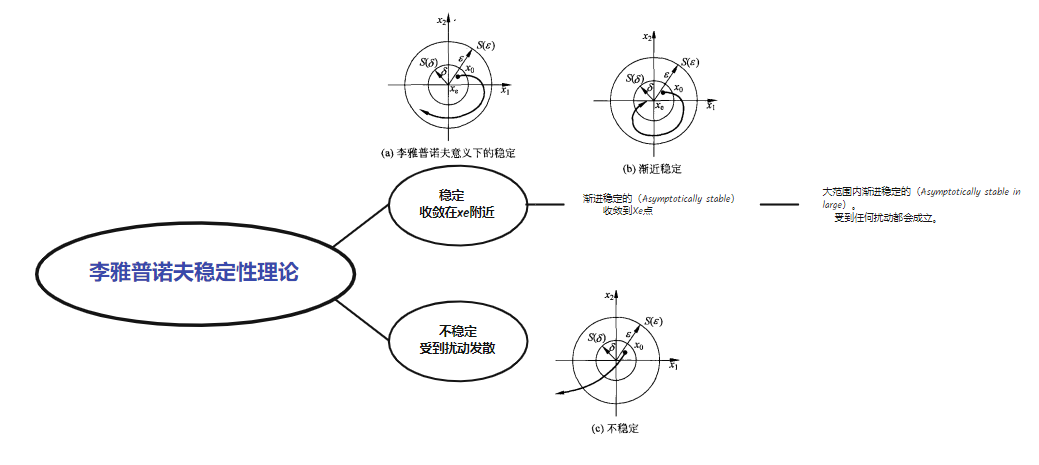工程上往往喜欢渐近稳定,因为希望干扰除去后,系统又会回到原来的工作状态,这个状态正是我们设计系统时所期望的,也就是前面所说的平衡状态。### 李雅普诺夫第二法

参考：李亚普诺夫函数
第二法就比较天才了，来源于一个朴素的想法：稳定的系统能量总是不断被耗散的，李雅普诺夫通过定义一个标量函数 V ( x ) V_{(x)} （通常能代表广义能量）来分析稳定性。这种方法的避免了直接求解方程，也没有进行近似线性化，所以也一般称之为直接法。如果标量函数 V ( x ) V_{(x)} 满足,则满足李雅普诺夫稳定性：则称系

可见，如果能合理的选定李雅普诺夫函数，则非常容易的判断系统的稳定性。不过遗憾的是，对于复杂的系统，李雅普诺夫函数的选择可以称得上一门玄学，所以，对于工程师而言，笔者还是喜欢李雅普诺夫第一法。

李氏第二法是从能量观点出发得来的，如果系统的总能量（含动能和势能）随时间增长而连续地衰减，直到平衡状态为止，那么振动系统是稳定的。

如果系统有一个渐近稳定的平衡状态，那么当它运动到平衡状态的邻域内时，系统积蓄的能量随时间的增长而衰减，直到平衡状态处达到最小值。李雅普诺夫引出了一个虚构的广义能量函数，这个函数具有能量的含义，但比能量更为一般，它有如下一些基本特征：

① 能量函数一定是状态变量x的函数。因为状态变量x可以对系统的动态行为进行完全描述，因此能量函数也一定是状态变量x的函数。

②V（x） 是正定

③ V（x）具有连续的一阶偏导数。

对于一个给定的系统，如果能找到一个正定的标量函数v(x)，直接利用及的v(x)及其导数数的符号特征判别出平衡状态处的稳定性，则这标量函数V（x）就称为李雅普诺夫函数。## 李雅普诺夫稳定（内部稳定）与BIBO稳定（外部稳定）的关系

即渐近稳定要求的条件严于BIBS稳定,而BIBS稳定要求的条件又严于BIBO稳定。但是,如果系统是李雅普诺夫意义下稳定的,则系统不一定是BIBS稳定的和BIBO稳定的。例如,系统

### BIBS稳定性 (Bounded-Input bounded-state)

(6) BIBS 稳定性。对任意有界的x(0),若在任意有界的输入u(t)的作用下,x(t)均有界,则称系统BIBS稳定。
x(t)均有界，内部稳定。
系统矩阵A的特征值全部位于左半复平面(不含虚轴)，则BIBS稳定。

### BIBO稳定性(Bounded Input Bounded Output)；

(7) BIBO稳定性。对任意有界的x(0),若在任意有界的输人u(t)的作用下,y(t)均有界,则称系统BIBO稳定。
外部稳定，y(t)均有界。
对于线性定常系统，若传递函数矩阵的极点全部位于左半复平面，则BIBO稳定。
为什么会定义李雅普诺夫稳定呢?因为BIBO稳定,就是从传递函数中得到的稳定性分析,是一种外部稳定,**有界输入可能会导致有界输出,但是此时系统内部不一定是稳定的,系统内部不稳定为什么外部会稳定呢?原因在于系统内部不稳定的子状态可能是不能观的。不能观的子状态无法从传递函数上反应出来。**在实际情况下,我们往往希望一个系统不仅仅是外部稳定的,最好还是内部稳定的。

参考：李雅普诺夫稳定（内部稳定）与BIBO稳定（外部稳定）的关系
参考：李雅普诺夫稳定性分析
线性定常系统BIBO稳定性
若传递函数矩阵的极点全部位于左半复平面，则BIBO稳定。
线性定常系统BIBS稳定性
系统矩阵A的特征值全部位于左半复平面，则BIBS稳定
对于线性定常系统，若系统是渐进稳定的，则必然BIBS和BIBO稳定
若BIBS，则BIBO
若李雅普诺夫意义下稳定，不一定是BIBS和BIBO

线性定常系统的BIBO稳定性判别主要依据传递函数矩阵进行,如果其极点全部位,于左半复平面(不含虚轴),则系统BIBO稳定。线性定常系统的BIBS稳定性判别主要依据系统矩阵A进行,如果其特征值全部位于左半复平面(不含虚轴),则系统BIBS稳定。对线性定常系统,如果系统是渐近稳定的,则系统必然是BIBS稳定的和BIBO稳定的。如果系统是BIBS稳定的,则系统必然是BIBO稳定的。即渐近稳定要求的条件严于BIBS稳定,而BIBS稳定要求的条件又严于BIBO稳定。但是,如果系统是李雅普诺夫意义下稳定的,则系统不一定是BIBS稳定的和BIBO稳定的。

## 系统的可控和可观测性

本质上是受限制与实际的物理的构成，FCU在自然条件下是会天然的不可被观测的。是否可控则需要看能否通过添加Input得到期望的映射关系。
8.3节介绍了系统的稳定性,本节介绍系统的另外两个重要属性,即系统的可控性和可观测性,这两个属性是经典控制理论中所没有的。在用传递函数描述的控制系统中,输出量一般是可控的和可以被测量的,因而不需要特别地提及可控性及可观测性的概念。现代控制理论用状态方程和输出方程描述系统,输出和输入构成系统的外部变量,而状态为系统的内部变量,系统就好比是一块集成电路芯片,内部结构可能十分复杂,物理量很多,而外部只有少数几个引脚,对电路内部物理量的控制和观测都只能通过这为数不多的几个引脚进行。这就存在着系统内的所有状态是否都受输入控制和所有状态是否都可以从输出反映出来的问题,这就是可控性和可观测性问题。如果系统所有状态变量的运动都可以通过有限的控制点的输人来使其由任意的初态达到任意设定的终态,则称系统是可控的,更确切地说是状态可控;否则,就称系统是不完全可控的,简称系统,不可控。相应地,如果系统所有状态变量的任意形式的运动均可由有限测量点的输出完全确定出来,则称系统是可观测的,简称系统可观测;反之,则称系统是不完全可观测的,称系统不可对
参考：[现代控制理论2-4(1)] 系统能控性分析
刘豹：在现代控制理论中，能控性和能观性是两个重要的概念，是卡尔曼（Kalman）在1960年首先提出来的，它是最优控制和最优估计的设计基础。前以指出现代控制理论是建立在用状态空间描述的基础上的。状态（微分）方程描述了输入 u ( t ) u_{(t)} 引起系统状态 x ( t ) x_{(t)} 的变化过程；输出方程则描述了由于系统状态变化引起的输出 y ( t ) y_{(t)} 的变化。
能控性是分析 u ( t ) u_{(t)} 对状态 x ( t ) x_{(t)} 的控制能力，能观性是分析输出 y ( t ) y_{(t)} 对状态 u ( t ) u_{(t)} 的反映能力。

## 1 可控性

为什么研究动力学系统的可控性？其实是研究 系统的输入 对于系统的 “塑造” 能力——对系统状态的影响，线给出系统能控性的定义：那么一句话说 可控性 研究的问题：给定终值（origin），询问是否存在合适的初始状态出发点（初值）使得能够设计一个 系统控制输入 u ( t ) u_{(t)} 来实现转移。
可控性分析也分为：

### 1 时间连续可控性当它的 状态点（Zustand punkt x ( t ) x_{(t)} ）能通过合适选择的 输入 [公式] 在 有限的步骤内（in endlich vielen Schritten）从任意的初始状态 (Anfangszustand) x 0 ) x_{0)} 到达 0 终点状态 （Endzustand x ( e ) = x ( 0 ) x_{(e)}=x_{(0)} 平衡点？），那么就称这个系统是 完全可控的（vollständig steuerbar）。

1. 有限步骤
2. 任意初始状态
3. 0 终点状态
除了 能控性（Steuerbarkeit），同时也定义 能达性（Erreichbarkeit），定义：比较下两个定义，简单来说，两者的所讨论的“方向”是相反的：
此 能达性强调的是：确定的起点/初始状态，探讨从起点出发后终值的最后去向。即我们更关心，从状态空间指定的某一点出发，最终能不能到达其他任意的地方。
能控性强调的是：能不能从状态空间的任意的起点/任意的初始状态，最终能达到指定的某一终点状态（Endzustand [公式] ）。

#### 可控性判据

1 图判断 见 练习 folie只有少数简单的系统可以从结构图或信号流图直接判别系统的可控性与可观测性,如果系统结构复杂,就只能借助于数学方法进行分析与研究,才能得到正确的结论。

##### 3 KALMAN: 将推导 Kalman 判据 最常见能控的充分必要条件是：系统的可控性矩阵满秩=秩为 n n## 2 可观性

展开全文linux
• 通过ode45方程求解洛仑兹系统，然后画混沌的分岔图，通过分岔图分析系统的混沌动力学行为。
• MATLAB常见非线性可视化绘制方法-分岔图与庞加莱截面（混沌可视化、Poincare截面、Logistic、Henon、Lorenz、Rossler、Duffing系统

### MATLAB常见非线性可视化绘制方法-分岔图与庞加莱截面（混沌可视化、Poincare截面、Logistic、Henon、Lorenz、Rossler、Duffing系统）

本文首发于 matlab爱好者 微信公众号，欢迎关注。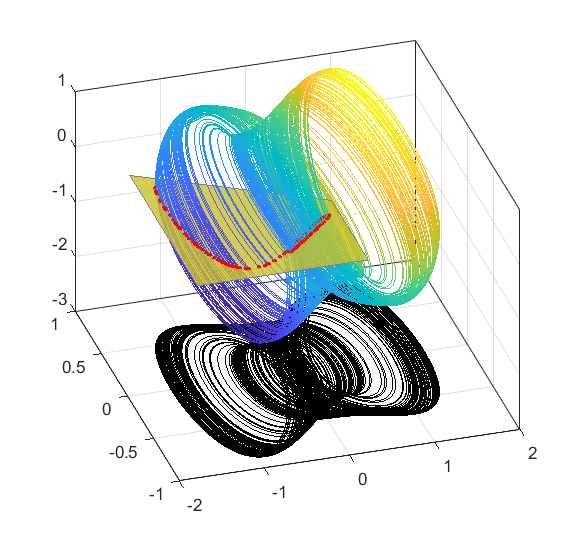惯例声明：本人没有相关的工程应用经验，只是纯粹对相关算法感兴趣才写此博客。所以如果有错误，欢迎在评论区指正，不胜感激。本文主要关注于算法的实现，对于实际应用等问题本人没有任何经验，所以也不再涉及。

# 1 引言

本文大概介绍一下常用的非线性可视化的方法，由于篇幅问题，分了两章。第一篇介绍一下相图与相空间的概念。第二篇介绍一下庞加莱截面和分岔图的绘制方法。后面第三篇就不打算写可视化了，看看要不要写一个频谱分析、李雅普诺夫指数分析、维数分析之类的时间序列分析，但这一部分有趣性不高，实在没动力o(╥﹏╥)o。

非线性的数学研究诞生时间还是比较久了，从蝴蝶效应开始一直到现在，常用的分析方法变化不是太大。各领域研究方向有所不同，但在论文中如果涉及到方程的非线性，总会需要定性的分析一下，由于方法比较类似，所以常常也可以参阅别的领域的非线性分析相关的方法或者好看的绘图方式。

这篇博客主要讲怎么绘制分岔图，顺便也提了一下怎么绘制庞加莱截面。分岔一般指的是系统中某一个常数发生变化，导致整个系统都会随之改变的现象。在电路中可能是某个电容改变，在机械系统中可能是某个轴承摩擦阻力的变化，在流场中可能是风速的改变。这些参数微小的变化，就会导致系统突然性情大变，变得震荡发散不收控制。

上一篇介绍了相图的绘制，这个可以看做是非线性可视化的基础，建议先看一眼上一篇文章：
MATLAB常见非线性可视化绘制方法-相图与相空间https://blog.csdn.net/weixin_42943114/article/details/123193855

参考目录
Morris W. Hirsch. 微分方程、动力系统与混沌导论[M]. 人民邮电出版社, 2008.
刘秉正. 非线性动力学与混沌基础[M]. 东北师范大学出版社, 1994.
 Khalil H K . 非线性系统(第3版)[M].电子工业出版社,2005.
Morris W. Hirsch. Differential equations, dynamical systems, and an introduction to chaos [M] Academic Press
 微分方程、动力系统与混沌导论[M]
 计算物理基础-第10章第77讲（北京师范大学）（中国大学MOOC）计算物理基础_北京师范大学_中国大学MOOC(慕课) (icourse163.org)
Computing accurate Poincaré maps[J]. PHYSICA D, 2002, 171(3):127-137.
Santo Banerjee. Applications of Chaos and Nonlinear Dynamics in Engineering[M]. Springer, 2011
Ali.H.Nayfeh. Applied Nonlinear Dynamics Analytical, Computational, and Experimental Methods[M]. 1995.(用到了P284里面的Henon系统)
詹姆斯·格雷克. 混沌：开创新科学[M].

# 2 离散非线性系统的分岔图绘制

## 2.1 一维Logistic系统分岔图

Logistic系统是一种非常简单的二次多项式形式的映射。该映射是1976 年生物学家罗伯特·梅 (Robert May) 发扬光大的，用来说明即使很简单的系统也可以产生混沌现象。其迭代方程如下：
x n + 1 = a ( 1 − x n ) x n x_{n+1}=a(1-x_n)x_n
这个迭代方程用来表述某个物种的数量增长变化。其中x的含义为现有物种数量除以理论最大数量，a为增长率。方程认为，物种数量的变化，与物种现存人口x有关，也与人口过多带来的环境压力(1-x)有关。

当增长率a小于1时，物种逐渐灭亡；当增长率在1-3之间，则物种能够稳定在一个范围内；当增长率更大，则会逐渐出现非线性现象。

绘制其分岔图有两种方法：

第一种，均匀分布初始点，然后进行逐次迭代，观察其运动规律。

比如下图选取a=2.1和a=3.3的两个参数，初始分布各个初值，进行迭代，观察这些初值的变化：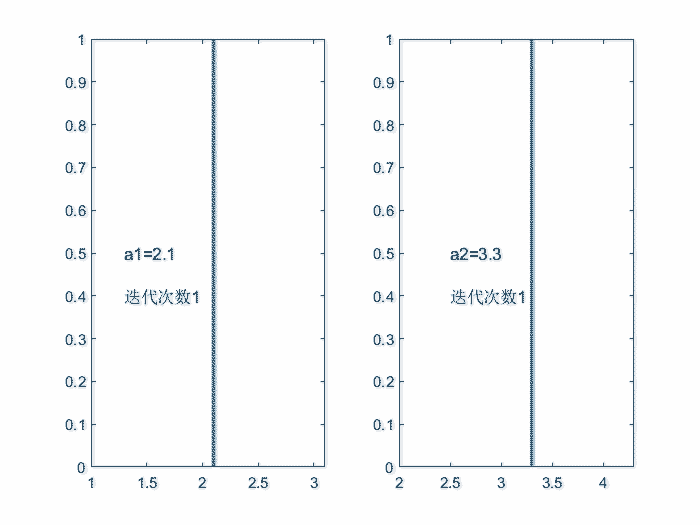可以看到当a=2.1时，最终所有种群收敛到了1个点。当a=3.3时，最终所有种群点收敛到了2个点。

继续细化a的值，列出一个图，可以看到不同参数a变化下，系统的收敛规律：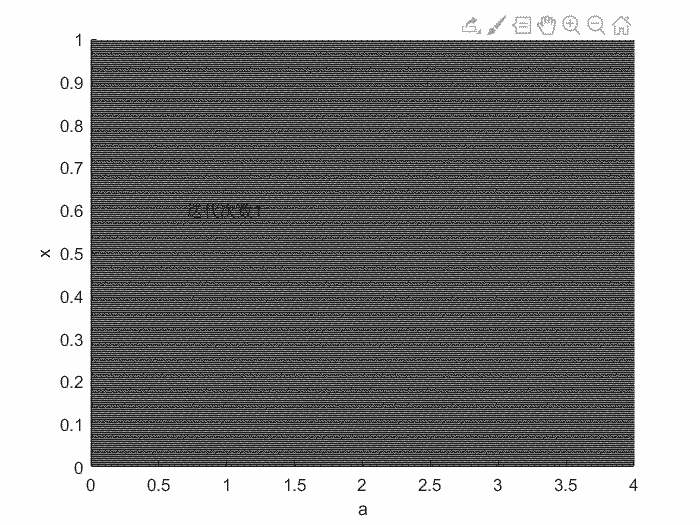最终迭代200次的结果如下图：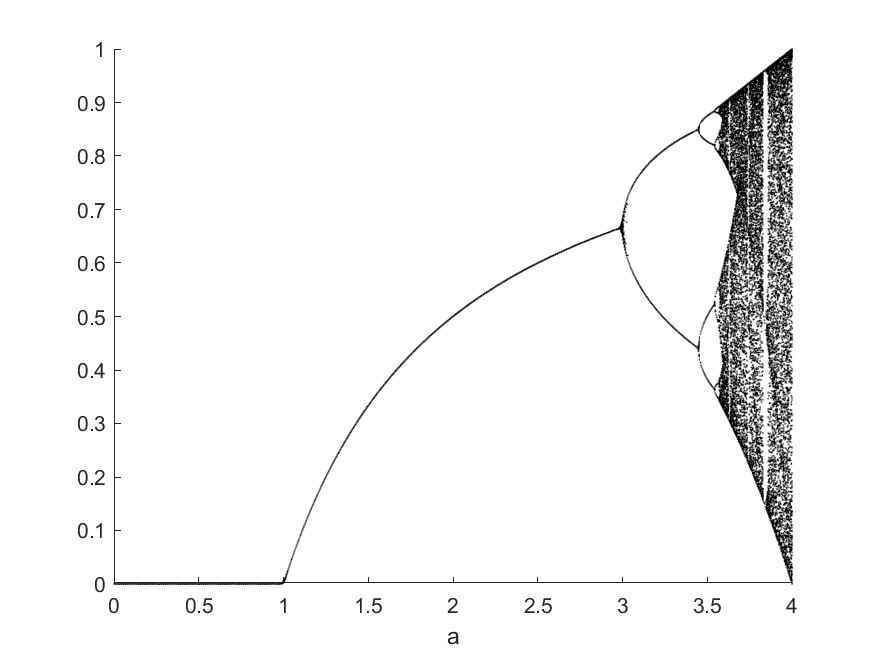这种体现随着某个参数（比如a）变化，导致系统行为发生改变的图，叫做分岔图

当然为了好看，也可以根据点的密度来加一点颜色，比如下面这个样子。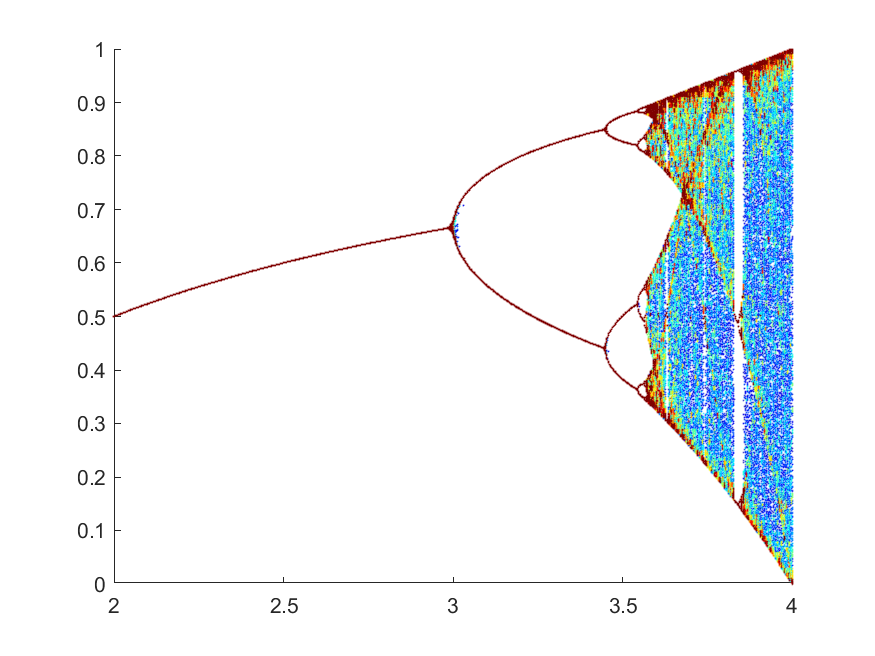上面几个图的代码如下：

clear
clc
close all
%分岔图
%离散系统

%% 1Logistic系统
%% 1.1Logistic系统 两个典型的a，进行迭代的效果
d=0.004;
x=d:d:1-d;
Nx=length(x);
BF=zeros(1,Nx);
a1=2.1;
a_k1=a1;%第一个a=2.1
a2=3.3;
a_k2=a2;%第二个a=3.3
x1=x;
x2=x;
%在系统中迭代50次
for m=1:50
%把结果绘图
f1=figure(1);
set(f1,'Color',[1,1,1])
clf
subplot(1,2,1)
plot(a1*ones(size(x1)),x1,'.')
ylim([0,1])
text(a1-0.8,0.5,['a1=',num2str(a1)])
text(a1-0.8,0.4,['迭代次数',num2str(m)])
subplot(1,2,2)
plot(a2*ones(size(x1)),x2,'.')
ylim([0,1])
text(a2-0.8,0.5,['a2=',num2str(a2)])
text(a2-0.8,0.4,['迭代次数',num2str(m)])
pause(0.1)
%更新下一次迭代
x1=Logistic(x1,a_k1);
x2=Logistic(x2,a_k2);
end

%% 1.2 不同a，绘制分岔图
%初始种群采用均匀分布
d=0.005;
x=d:d:1-d;
a=0:0.004:4;%把a采集的足够密，就可以绘制随参数a变化的分岔图

Nx=length(x);
Na=length(a);
BF=zeros(Na,Nx);%初始化最终储存的矩阵

for k=1:Na
a_k=a(k);
x1=x;
%在系统中迭代200次
for m=1:200
x1=Logistic(x1,a_k);
end
%把结果保存
BF(k,:)=x1;
end

%画图
figure()
hold on
for k=1:Na
a_k=a(k);
plot(a_k*ones(1,Nx),BF(k,:),...
'LineStyle','none','Marker','.','MarkerFaceColor','k','MarkerEdgeColor','k',...
'MarkerSize',1)
end
hold off
xlabel('a')

%% 1.3 不同a 动图，把上面那个分岔图的过程展示出来
d=0.005;
x=d:d:1-d;
a=0:0.004:4;

Nx=length(x);
Na=length(a);
BF=ones(Na,1)*x;
BFx=a'*ones(1,Nx);
%在系统中迭代100次
for m=1:90
%绘制图片
f3=figure(3);
clf
scatter(BFx(:),BF(:),0.5,'k','MarkerEdgeAlpha',0.5)
text(0.7,0.6,['迭代次数',num2str(m)])
xlabel('a')
ylabel('x')
set(f3,'Color',[1,1,1])
pause(0.1)

%迭代更新
for k=1:Na
a_k=a(k);
x1=BF(k,:);
x1=Logistic(x1,a_k);
%把结果保存
BF(k,:)=x1;
end
end

%% 1.4 不同a上颜色
d=0.002;
x=d:d:1-d;
a=0:0.002:4;
Nx=length(x);
Na=length(a);
BF=zeros(Na,Nx);

for k=1:Na
a_k=a(k);
x1=x;
%在系统中迭代250次
for m=1:250
x1=Logistic(x1,a_k);
end
%把结果保存
BF(k,:)=x1;
end
%上颜色
BF_C=zeros(size(BF));
for k=1:Na
BF_k=BF(k,:);
[N,~,bin] = histcounts(BF_k,[0:0.01:1]);%统计每个小区间，点的数量，作为颜色
BF_C(k,:)=N(bin);%记录各个点的颜色
end
BFy=BF;
BFx=a'*ones(1,Nx);
figure()
scatter(BFx(:),BFy(:),0.5,BF_C(:),'MarkerEdgeAlpha',0.5)
caxis([0,20])
colormap(jet)
ylim([0,1]);
xlim([2,4]);

%% 后置函数
function x2=Logistic(x1,a)
%Logistic系统
%x(n+1)=a*(1-x(n))*x(n)
x2=a*(1-x1).*x1;
end


前面说分岔图绘制方法有两种，那么第二种，就是选取一个随机的初始点进行迭代，然后取它最后200次的历史位置（或者说运动过程），作为分岔图，而不是最终时刻的所有点的所有位置。

这种方式的优点是计算快，初始点只选取一个就可以，不需要上面动用200个点一起迭代。

代码如下，具体代码差别可以和上面方法1中的代码1.2进行对比：

%% 2.1 换一种计算方法
%初始化
a=0:0.004:4;
Nx=200;%最终储存最后200个点
Na=length(a);
BF=zeros(Na,Nx);

for k=1:Na
a_k=a(k);
x0=0.234;%随便初始一个点
for m=1:100 %先初始100步，来排除初始点选取的干扰
x0=Logistic(x0,a_k);
end
xs=zeros(1,Nx);%再正式开始循环，记录下一个点在迭代时候每一个位置
for m=1:Nx
x2=x0;
x0=Logistic(x2,a_k);
xs(m)=x2;
end
%把结果保存
BF(k,:)=xs;
end
%画图%这里用scatter还是plot函数绘图都行
figure()
hold on
for k=1:Na
a_k=a(k);
plot(a_k*ones(1,Nx),BF(k,:),...
'LineStyle','none','Marker','.','MarkerFaceColor','k','MarkerEdgeColor','k',...
'MarkerSize',1)
end
hold off
xlabel('a')
ylabel('x')

%% 后置函数
function x2=Logistic(x1,a)
%Logistic系统
%x(n+1)=a*(1-x(n))*x(n)
x2=a*(1-x1).*x1;
end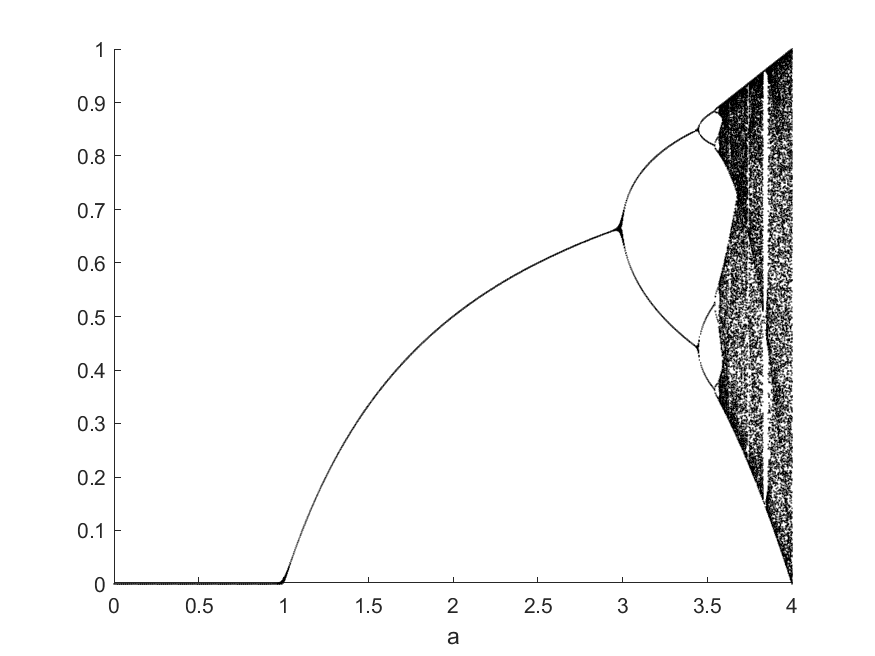最终结果如上，可以看到和前面方法一的结果相差不大。

因为该收敛的终究会收敛，该分岔的也会分岔，该混沌的依然会混沌，这是系统自己的行为特性，和初始选取的点无关。

另外，再多啰嗦一句，Logistic系统有一个非常典型的倍周期分岔特性，也就是分岔图的那根线，由1个分成了2个，2个分成了4个，4个又变成了8个，然后分岔的速度越来越快，直到进入混沌状态。这里2个岔代表在迭代结果在两个点上来回变动，周期为2；同理4个岔代表点在4个点上来回跳动，周期为4。这就是我个人对倍周期分岔的一个比较形象的解释。

## 2.2 二维Henon系统分岔图

Henon系统由 Michel Hénon大概在20世纪六七十年代，作为Lorenz模型的 Poincaré截面的简化模型引入。因此，我也准备将其作为衔接离散系统与庞加莱截面的一个关键切入口。

所以这一小节，用来如何用二维离散系统来绘制分岔图。

Henon系统有两个变量x和y，其迭代公式为：
x n + 1 = 1 + y n − a ∗ x n 2 y n + 1 = b ∗ x n x_{n+1}=1+y_n-a*x_n^2 \\ y_{n+1}=b*x_n
这里我们取b=0.3，则系统的可变参数只剩下a。

当a=0.2时，整个系统最终稳定到（x=1.09,y=3.27）这个点上。当a=0.95时，系统则在4个点上来回周期运动。当a=1.38时，系统则会陷入混沌。

下图可以看到当取不同a值时，系统的最终形式。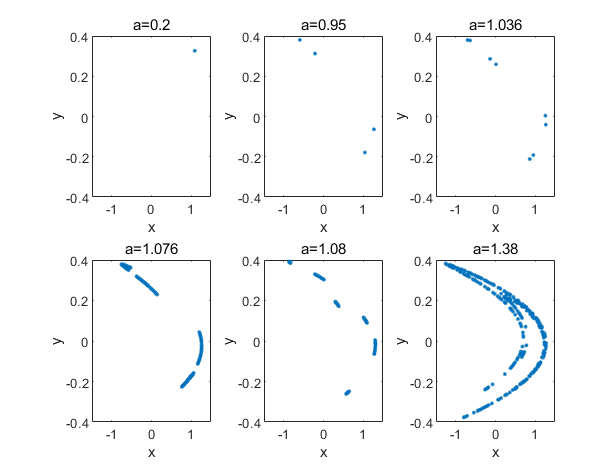那么如何将这个二维的结果图转换到分岔图上呢？在前面一维的Logistic系统分岔图中，纵轴直接就是x。而对于二维离散结果，我们需要先投影到一维结果上，再绘制分岔图。

简单一点，可以直接投影的y轴上，我们用系统的y值来绘制分岔图；或者投影到x轴上，用系统的x值绘制分岔图。（或者投影到某个特殊的线上，不过一般简单点就直接投影到轴上了）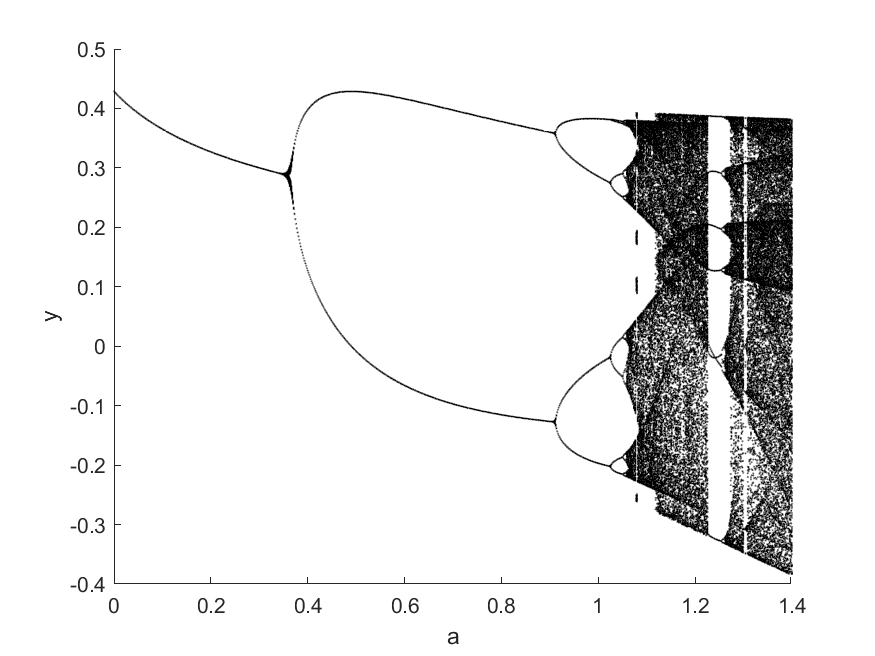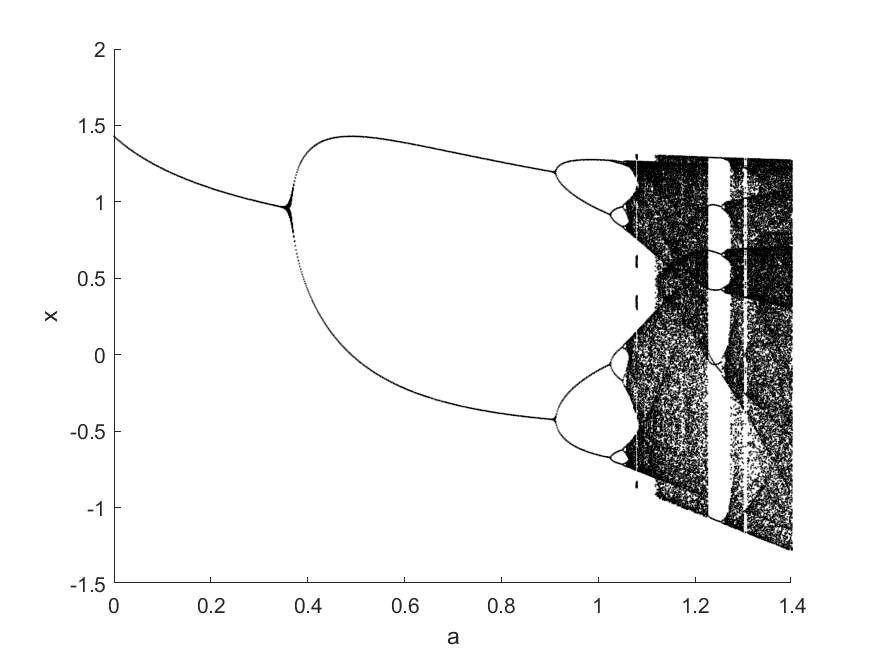可以看到，除了形状可能会稍微有点不同，其分岔点的位置和分岔后的形态是完全一致的。
因此，对于二维系统或更高维系统的分岔图，只需要把离散点投影到一个轴上就可，不影响系统分岔点的分析和判断。

绘图代码如下。这里由于二维计算量较大，所以直接用的是第二种方法，也就是只选取一个初始点进行迭代，然后记录过程。

clear
clc
close all
%分岔图
%离散系统
%% 2 Henon系统
a=0:0.0016:1.4;
%采用直接截取迭代中间过程，作为分岔图
NT=300;%选取最后300次结果
Na=length(a);
BF_x=zeros(Na,NT);
BF_y=zeros(Na,NT);

for k=1:Na
a_k=a(k);
%初始值取0
x1=0;
y1=0;
%先初始迭代200次，避免初始值选取引起的干扰
for m=1:200
[x1,y1]=Henon(x1,y1,a_k,0.3);
end
%然后再迭代300次，作为要保存的结果
for m=1:NT
[x1,y1]=Henon(x1,y1,a_k,0.3);
%把结果保存
BF_x(k,m)=x1;
BF_y(k,m)=y1;
end
end

%分岔图1，用y绘图
figure()
hold on
for k=1:Na
a_k=a(k);
plot(a_k*ones(1,NT),BF_y(k,1:NT),...
'LineStyle','none','Marker','.','MarkerFaceColor','k','MarkerEdgeColor','k',...
'MarkerSize',1)
end
hold off
xlabel('a')
ylabel('y')
%分岔图2，用x绘图
figure()
hold on
for k=1:Na
a_k=a(k);
plot(a_k*ones(1,NT),BF_x(k,1:NT),...
'LineStyle','none','Marker','.','MarkerFaceColor','k','MarkerEdgeColor','k',...
'MarkerSize',1)
end
hold off
xlabel('a')
ylabel('x')
ylim([-1.5,2])
%绘制典型情况的最终x-y图
figure()
aa=[0.2,0.95,1.036,1.076,1.08,1.38];%要展示的aa图
for k=1:6
subplot(2,3,k)
[~,Ind_a]=min(abs(a-aa(k)));
plot(BF_x(Ind_a,:),BF_y(Ind_a,:),'LineStyle','none','Marker','.')
xlim([-1.5,1.5])
ylim([-0.4,0.4])
xlabel('x');ylabel('y');
title(['a=',num2str(aa(k))])
end

%% 后置函数
function [x2,y2]=Henon(x1,y1,a,b)
%Henon系统
%x(n+1)=1+y(n)-a*x(n)^2
%y(n+1)=b*x(n)
x2=1+y1-a*x1.*x1;
y2=b*x1;
end


# 3 庞加莱截面

## 3.1 绘制思路

对于一个混沌系统，其轨线往往是混乱无序的，很难去简洁的描述。那么其中一个思路就是，简化信息，降低描述的维度，把空间的轨线化简为一系列离散点。

对于一个周期或拟周期运动的系统，在相空间的运动表现为一圈又一圈的转动。我们定义一个截面（一般是平面），当轨线穿过这个面时，把交点记录下来。当记录足够多的交点后，这些交点形成的图像就是庞加莱截面的图像。我们把这个和轨线相交的面称作庞加莱截面（Poincaré section）。

接下来就以杜芬（Duffing）方程分岔图的绘制大概演示一下上面所说的大概思路。

Duffing方程是以 Georg Duffing命名的一个非线性方程。它是基于强迫振动的单摆所提出的方程，它提出的时间非常早，但是被拿来做混沌研究还是比较晚的。由于它背后有着非常明显与简单的物理模型，所以甚至可以做实验去观察这个方程的非线性。方程的形式为：

x ¨ = − x 3 + x − δ x ˙ + γ cos ⁡ ( ω t ) \ddot{x}=-x^3+x-\delta \dot{x}+\gamma \cos{(\omega t)}

把这个方程整理成一个三阶的方程组，得到：

[ x x ˙ z ] ′ = [ x ˙ − x 3 + x − δ x ˙ + γ z − ω sin ⁡ ( ω t ) ] \begin{bmatrix} x \\ \dot{x} \\ z \end{bmatrix} ' =\begin{bmatrix} \dot{x} \\ -x^3+x-\delta \dot{x}+\gamma z \\ -\omega \sin{(\omega t)} \end{bmatrix}
这里有个随时间变化的变量cos(wt)，为了方便分析，把它单独定义为z=cos(wt)。

现在，我就得到了一个三阶的非线性方程组。默认ω=1，γ=1。

之后，我们定义空间中的z=0作为其要切割轨线的平面。而且当轨线正向穿过平面时才被记录，反向穿过平面则不被记录。这是为了让一个周期只取记录1个点。

下图是当δ=1.5、δ=1.35、δ=1.15时，其三维轨线和庞加莱截面：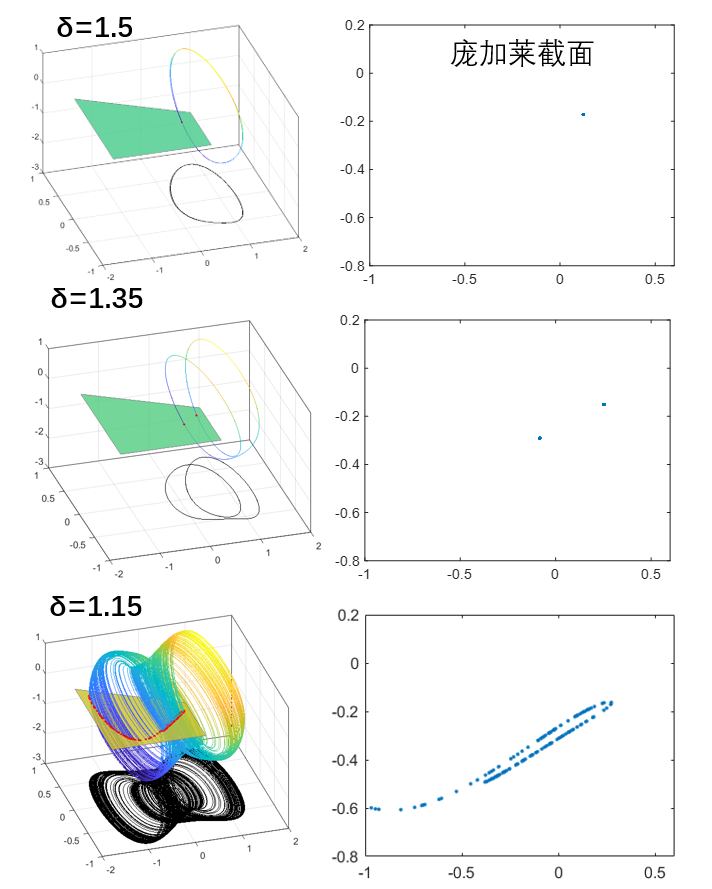当然，实际上定义的z=0截面应该是空间上没有边界限制无穷大的，这里为了方便作图，所以用了一个四边形来代替，希望不要造成误解。

通过庞加莱截面，就可以知道系统的大概特性。比如当δ=1.5时，庞加莱截面是只有1个点，证明系统是周期1的系统。当δ=1.35时，庞加莱截面是变为2个点，说明系统是周期2的系统，每转两次回到起点。当δ=1.5时，庞加莱截面是变为接近一条曲线，说明系统很可能是一个准周期运动。

所以庞加莱截面的绘制方法可以分为3步：

1 计算出轨线
2 计算轨线与某一平面的交点
3 将交点绘制出来，就得到了庞加莱截面


其中第二步计算交点，通常采用插值的方法得到。

这里给出绘图代码：

%庞佳莱截面
%截面采用公式Ax+By+Cz+D=0;的形式
%采用杜芬方程
clear
clc
close all
%% 第一步，计算出轨迹
h=5e-3;
x0=0:h:1600;
y0=[0.1;0.1;1];%最后一项是cos(w*t)，当t=0时必须为1.
[y1,Output]=ODE_RK4_hyh(x0,h,y0,[1.5,1,1]);
%[1.5,1,1],[1.35,1,1],[1.15,1,1]
Lx=y1(1,2000:end);
Ly=y1(2,2000:end);
Lz=y1(3,2000:end);
%% 第二步，计算与平面的交点
Plane=[0;0;1;0];%一般情况下是个垂直某个轴的平面。这里是Ax+By+Cz+D=0的形式给出的平面。
[tP_List,yP_List]=Solve_Poincare(x0,y1,Plane);%计算Poincare平面

%% 第三步，绘制庞加莱截面
%1庞加莱截面
%最开始几个点还没有稳定，没有体现出系统特点，所以放弃
figure()
plot(yP_List(1,10:end),yP_List(2,10:end),'.')%从第10个点之后开始绘制
xlim([-1,0.6])
ylim([-0.8,0.2])

%2展示用的示意图，只作为演示
figure()
hold on
patch([Lx,nan],[Ly,nan],[Lz,nan],[Lx+Ly,nan],...
'EdgeColor','interp','Marker','none','MarkerFaceColor','flat','LineWidth',0.8,'FaceAlpha',1);
plot3(yP_List(1,10:end),yP_List(2,10:end),zeros(size(yP_List(2,10:end))),...
'.','MarkerSize',8,'color','r')
patch([-1.6,0.4,0.4,-1.6],[-0.7,-0.7,0.0,0.6],[0,0,0,0],[1,1,1,1],...
'FaceAlpha',0.8,'EdgeColor',[0.5,0.5,0.5])
view([-17,39])
box on
grid on
%绘制投影的相图
set(gcf,'position',[300 200 560 500])
xlim([-2,2])
zlim([-3,1])
plot3( Lx,Ly,zeros(size(Ly))-3 ,'color','k')
hold off

%% 后置函数
function [tP_List,yP_List]=Solve_Poincare(t,y,Plane)
%截面方程z=0
% Plane=[0;0;1;0];%一般情况下是个垂直某个轴的平面
%一般只记录从负到正穿越。如果想反向也记录，可以设置Plane=-Plane。

%第二步，插值得到线与面的交点
yP_List=[];
tP_List=[];
Dis=DistancePlane(y,Plane);
N=size(y,2);
for k=1:N-1
if Dis(k)<=0 && Dis(k+1)>0
t0=t(k);t1=t(k+1);
yP0=y(:,k);yP1=y(:,k+1);
Dis0=Dis(k);Dis1=Dis(k+1);
%一维线性插值，求Dis=0时的t和y
%（相比较前面积分的4阶RK，这里用线性插值精度有点低，先这么凑合着吧）
yP=yP0+(yP1-yP0)/(Dis1-Dis0)*(0-Dis0);
tP=t0+(t1-t0)/(Dis1-Dis0)*(0-Dis0);
%储存
yP_List=[yP_List,yP];
tP_List=[tP_List,tP];
end
end
end

%点到平面的距离
function Dis=DistancePlane(xk,Plane)
% xk，坐标点，如果是3维坐标，大小就是3*N的矩阵。
% Plane，平面，形如Ax+By+Cz+D=0形式的平面。

N=size(xk,2);%计算总共多少个点
xk2=[xk;ones(1,N)];
Dis=dot(xk2,Plane*ones(1,N),1)./norm(Plane(1:end-1));
end

%两点线性插值
function y=interp2point_linear(x0,x1,y0,y1,x)
y=y0+(y1-y0)/(x1-x0)*(x-x0);
end

%两点3次插值
function y=interp2point_spline(x0,x1,y0,y1,x)
%y0包含y0的值和y0的导数,yy=y0(1),dy=y0(2)
xx0=x0;
xx1=x1;
yy0=y0(1);dy0=y0(2);
yy1=y1(1);dy1=y1(2);
cs = csape([xx0,xx1],[dy0,yy0,yy1,dy1],[1,1]);
y=ppval(cs,x);
end

function [F,Output]=Fdydx(x,y,Input)
%形式为Y'=F(x,Y)的方程，参见数值分析求解常系数微分方程相关知识
%高次用列向量表示，F=[dy(1);dy(2)];y(1)为函数，y(2)为函数导数
%杜芬方程duffing，参见中国大学MOOC，北京师范大学-计算物理基础-77倒摆与杜芬方程
d=Input(1);
r=Input(2);
w=Input(3);

dy(1)=y(2);
dy(2)=-y(1)^3+y(1)-d*y(2)+r*y(3);
dy(3)=-w*sin(w*x);

F=[dy(1);dy(2);dy(3)];
Output=[];
end

function [y,Output]=ODE_RK4_hyh(x,h,y0,Input)
%4阶RK方法
%h间隔为常数的算法
y=zeros(size(y0,1),size(x,2));
y(:,1)=y0;
for ii=1:length(x)-1
yn=y(:,ii);
xn=x(ii);
[K1,~]=Fdydx(xn    ,yn       ,Input);
[K2,~]=Fdydx(xn+h/2,yn+h/2*K1,Input);
[K3,~]=Fdydx(xn+h/2,yn+h/2*K2,Input);
[K4,~]=Fdydx(xn+h  ,yn+h*K3  ,Input);
y(:,ii+1)=yn+h/6*(K1+2*K2+2*K3+K4);
end
Output=[];
end


## 3.2 利用频闪法快速绘制

这种计算方法，具体思路还是那三步。

1 计算出轨线
2 计算轨线与某一平面的交点
3 将交点绘制出来，就得到了庞加莱截面


但是第二步不再是直接通过轨线上两点进行插值得到，而是直接通过时间t得到。

比如下面是我们3.1节的杜芬方程，我们取的是z=0平面作为庞加莱截面。
[ x x ˙ z ] ′ = [ x ˙ − x 3 + x − δ x ˙ + γ z − ω sin ⁡ ( ω t ) ] \begin{bmatrix} x \\ \dot{x} \\ z \end{bmatrix} ' =\begin{bmatrix} \dot{x} \\ -x^3+x-\delta \dot{x}+\gamma z \\ -\omega \sin{(\omega t)} \end{bmatrix}
但是，z=0明明是可以计算出来的。z=cos(t)，当t=0.5π+2kπ和t=1.5π+2kπ的时候，z无条件的等于0。
对于庞加莱截面，一个周期我们只取一个点，所以所有位于庞加莱截面上的点，其时间t都应该满足t=1.5π+2kπ。

因为，对于频闪采样法，意思就是隔一段时间取一次点，这些点天然的在某个庞加莱截面上。这种方法采用预先计算得到的时刻间隔，大大减少了程序内的计算量。

同样取δ=1.15，其庞加莱截面的结果如下图。可以看到这种偷懒方法和上一章节老老实实计算结果相同。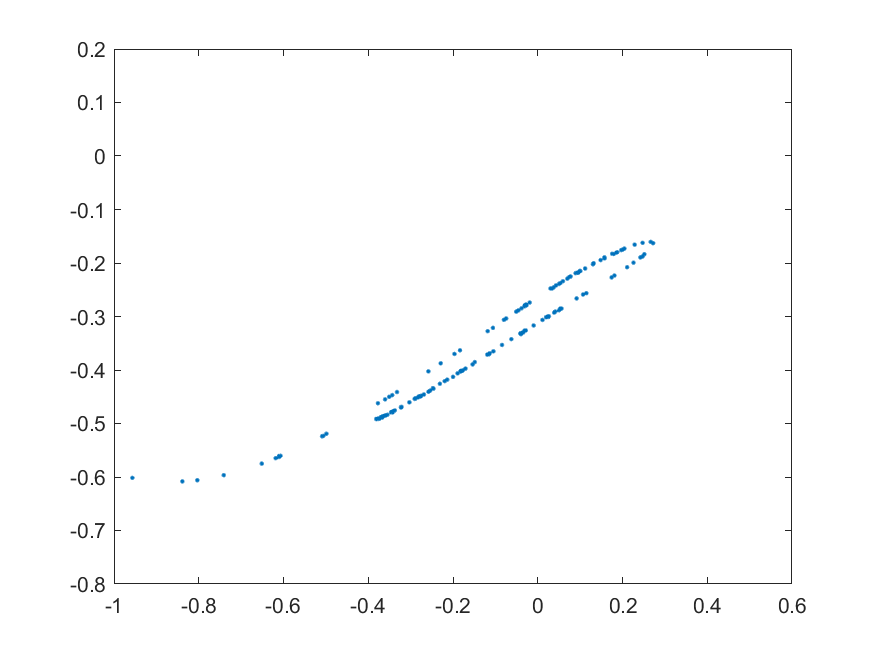事实上，由于不需要再计算z具体的值，方程中甚至可以省略掉计算z的步骤。这样就变成了虽然没有z这变量，但是却依然计算了系统在z=0的庞加莱截面。
[ x x ˙ ] ′ = [ x ˙ − x 3 + x − δ x ˙ + γ cos ⁡ ( ω t ) ] \begin{bmatrix} x \\ \dot{x} \\ \end{bmatrix} ' =\begin{bmatrix} \dot{x} \\ -x^3+x-\delta \dot{x}+\gamma \cos{(\omega t) } \end{bmatrix}
代码如下：

%庞佳莱截面（频闪法）
%采用杜芬方程
clear
clc
close all
%第一步，计算出轨迹
h=5e-3;
x0=0:h:1600;
y0=[0.1;0.1];%这里简化了方程，所以只有两个输入
[y1,Output]=ODE_RK4_hyh(x0,h,y0,[1.15,1,1]);
%[1.5,1,1],[1.35,1,1],[1.15,1,1],[0.1,0.35,1.4]
Lx=y1(1,:);
Ly=y1(2,:);
%Lz=cos(1*x0);

%采用频闪采样法计算
tP_Ideal=3*pi/2:(2*pi/1):x0(end);%这里考虑z=0平面，时间间隔dt=2*pi。
tP_List=zeros(1,length(tP_Ideal));
Ind_List=zeros(1,length(tP_Ideal));
for k=1:length(tP_Ideal)
[~,Ind]=min(abs( tP_Ideal(k)-x0 ));%直接根据索引来找到对应的点
Ind_List(k)=Ind;
tP_List(k)=x0(Ind);
end
yP_List=y1(:,Ind_List);
%注，如果上面时间间隔h为pi/600之类的形式，这里连min函数都可以取消，直接按照索引去寻找就行

%绘图
%1庞加莱截面
%最开始几个点还没有稳定，没有体现出系统特点，所以放弃
figure()
plot(yP_List(1,10:end),yP_List(2,10:end),'.')
xlim([-1,0.6])
ylim([-0.8,0.2])

function [F,Output]=Fdydx(x,y,Input)
%形式为Y'=F(x,Y)的方程，参见数值分析求解常系数微分方程相关知识
%高次用列向量表示，F=[dy(1);dy(2)];y(1)为函数，y(2)为函数导数
d=Input(1);
r=Input(2);
w=Input(3);

dy(1)=y(2);
dy(2)=-y(1)^3+y(1)-d*y(2)+r*cos(w*x);
% dy(3)=-w*sin(w*x);%由于无需计算具体的z值，所以这里把z合并到了dy(2)项里，减少计算量

F=[dy(1);dy(2)];
Output=[];
end

function [y,Output]=ODE_RK4_hyh(x,h,y0,Input)
%4阶RK方法
%h间隔为常数的算法
y=zeros(size(y0,1),size(x,2));
y(:,1)=y0;
for ii=1:length(x)-1
yn=y(:,ii);
xn=x(ii);
[K1,~]=Fdydx(xn    ,yn       ,Input);
[K2,~]=Fdydx(xn+h/2,yn+h/2*K1,Input);
[K3,~]=Fdydx(xn+h/2,yn+h/2*K2,Input);
[K4,~]=Fdydx(xn+h  ,yn+h*K3  ,Input);
y(:,ii+1)=yn+h/6*(K1+2*K2+2*K3+K4);
end
Output=[];
end


这种方法只在某些特殊的存在明确周期性的系统中才可以用的到。

当[δ，γ，ω] = [ 0.1 ,0.35 ,1.4]时，系统会由于混沌，其庞加莱截面还会出现混沌形式：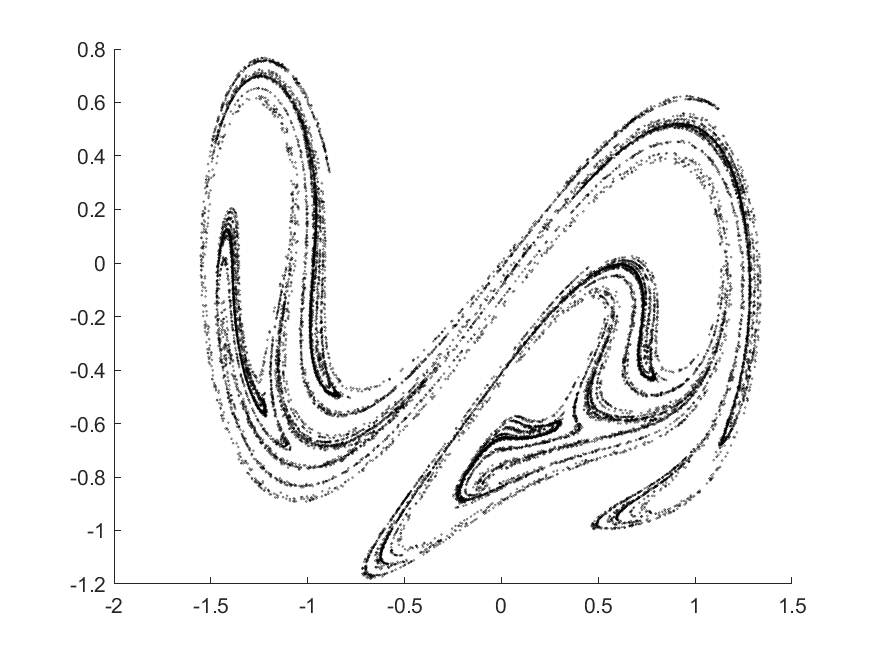上面的图涉及到的点非常多，所以无法一口气全部得到，只能采取一段一段计算的方式得到。绘图代码如下，仅供参考：

clear
clc
close all
%初始化
h=2*pi/700;
x0=0:h:2e5*pi/700;
y0=[0.1;0.1];%最后一项是cos(w*t)，当t=0时必须为1
%这里一口气求出一大堆点不太现实，内存有限，所以采取分批计算，逐步绘图来实现
figure(1)
hold on
for k=1:100
[y1,Output]=ODE_RK4_hyh(x0,h,y0,[0.1,0.35,1.4]);
Lx=y1(1,:);
Ly=y1(2,:);
%采用频闪采样法计算
%由于w变为了1.4，这里还是z=0平面，采样间隔改为 (3*pi/2:(2*pi/1):2000)/1.4
yP_List=y1(:,376:500:end);%注，这里376=3/2/1.4*350+1

%内存有点不够了，分段进行下面的计算
x0=x0+2e5*pi/700;%时间整体向后移动
y0=y1(:,end);%把最后一刻的状态当做下一个时间段初始状态
%绘图
%1庞加莱截面
scatter(yP_List(1,1:end),yP_List(2,1:end),0.8,'k','MarkerEdgeAlpha',0.6)
%disp(k)
end

function [F,Output]=Fdydx(x,y,Input)
%形式为Y'=F(x,Y)的方程，参见数值分析求解常系数微分方程相关知识
%高次用列向量表示，F=[dy(1);dy(2)];y(1)为函数，y(2)为函数导数
d=Input(1);
r=Input(2);
w=Input(3);
dy(1)=y(2);
dy(2)=-y(1)^3+y(1)-d*y(2)+r*cos(w*x);
F=[dy(1);dy(2)];
Output=[];
end

function [y,Output]=ODE_RK4_hyh(x,h,y0,Input)
%4阶RK方法
%h间隔为常数的算法
y=zeros(size(y0,1),size(x,2));
y(:,1)=y0;
for ii=1:length(x)-1
yn=y(:,ii);
xn=x(ii);
[K1,~]=Fdydx(xn    ,yn       ,Input);
[K2,~]=Fdydx(xn+h/2,yn+h/2*K1,Input);
[K3,~]=Fdydx(xn+h/2,yn+h/2*K2,Input);
[K4,~]=Fdydx(xn+h  ,yn+h*K3  ,Input);
y(:,ii+1)=yn+h/6*(K1+2*K2+2*K3+K4);
end
Output=[];
end


# 4 非线性系统的分岔图

前面介绍了离散系统绘制分岔图。对于连续系统，如何绘制分岔图呢？

大概思路为：

  1先绘制出系统的轨线 （3维系统转3维曲线，用轨线）
2绘制轨线和某个面的交点（3维曲线转2维点，用庞加莱截面）
得到某个平面的上的若干点后，问题就转化为前面二维离散系统的分岔图了：
4将这些点投影到某个线上（2维的点转1维的值）
5绘制分岔图


目的就是把连续系统转化为可以绘制分岔图的散点形式。

## 4.1 Duffing系统分岔图

以前面的介绍的杜芬方程为例，固定ω=1，γ=1，改变δ的分岔图如下。绘制方法就是计算出每一个δ下的庞加莱截面，然后仿照前面离散点分岔的，取坐标y作为分岔图。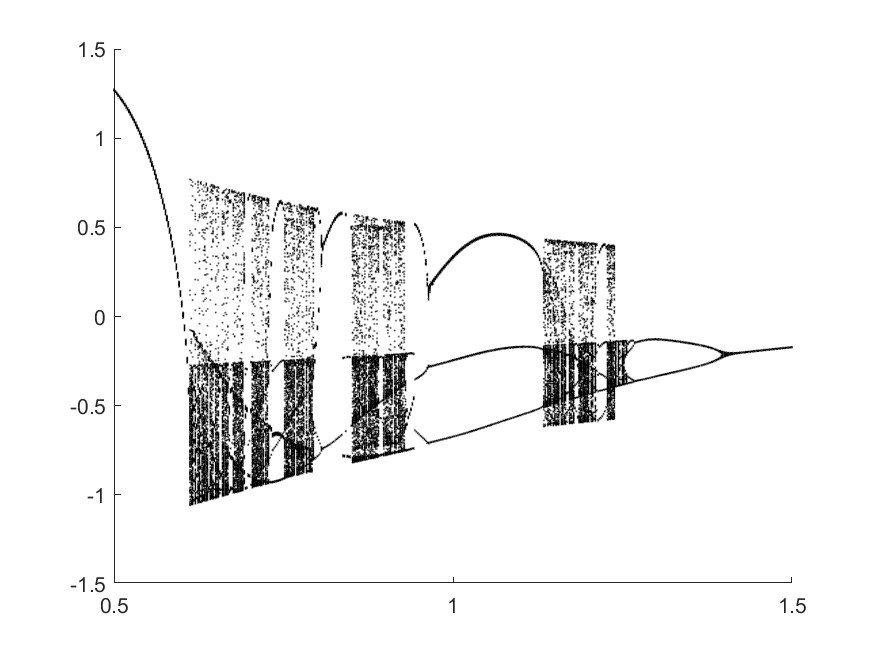绘图代码如下，计算时间比较长，需要耐心等待一段时间：

%利用庞佳莱截面绘制分岔图
clear
clc
close all
%第一步，计算出轨迹
h=8e-3;
x0=0:h:1600;
y0=[0.1;0.1];%最后一项是cos(w*t)，当t=0时必须为1.

%不同的d
d=0.5:0.002:1.5;%0.02
N_c=length(d);
N_P=250;%假设穿过截面的共有250个点
BF=nan(N_c,N_P);
%第二步，这里直接用频闪法求出截面所在点的索引
%z=0平面
tP_Ideal=3*pi/2:(2*pi/1):x0(end);%这里考虑z=0平面，时间间隔dt=2*pi。
tP_List=zeros(1,length(tP_Ideal));
Ind_List=zeros(1,length(tP_Ideal));
for m=1:length(tP_Ideal)
[~,Ind]=min(abs( tP_Ideal(m)-x0 ));
Ind_List(m)=Ind;
tP_List(m)=x0(Ind);
end
%第三步，开始对每一个d进行循环，计算其庞加莱截面
for k=1:N_c
d_k=d(k);
[y1,~]=ODE_RK4_hyh(x0,h,y0,[d_k,1,1]);
yP_List=y1(:,Ind_List);
%储存
N_P_temp=size(tP_List,2);
if N_P_temp>N_P
BF(k,1:N_P)=yP_List(2,1:N_P);%取坐标y作为分岔图
else
BF(k,1:N_P_temp)=yP_List(2,1:N_P_temp);%取坐标y作为分岔图
end
disp(d_k)
end
%最后一步，绘图
figure()
hold on
for k=1:N_c
d_k=d(k);
plot(d_k*ones(1,N_P-30+1),BF(k,30:N_P),...
'LineStyle','none','Marker','.','MarkerFaceColor','k','MarkerEdgeColor','k',...
'MarkerSize',1)
end
hold off

function [F,Output]=Fdydx(x,y,Input)
%形式为Y'=F(x,Y)的方程，参见数值分析求解常系数微分方程相关知识
%高次用列向量表示，F=[dy(1);dy(2)];y(1)为函数，y(2)为函数导数
d=Input(1);
r=Input(2);
w=Input(3);
dy(1)=y(2);
dy(2)=-y(1)^3+y(1)-d*y(2)+r*cos(w*x);
F=[dy(1);dy(2)];
Output=[];
end

function [y,Output]=ODE_RK4_hyh(x,h,y0,Input)
%4阶RK方法
%h间隔为常数的算法
y=zeros(size(y0,1),size(x,2));
y(:,1)=y0;
for ii=1:length(x)-1
yn=y(:,ii);
xn=x(ii);
[K1,~]=Fdydx(xn    ,yn       ,Input);
[K2,~]=Fdydx(xn+h/2,yn+h/2*K1,Input);
[K3,~]=Fdydx(xn+h/2,yn+h/2*K2,Input);
[K4,~]=Fdydx(xn+h  ,yn+h*K3  ,Input);
y(:,ii+1)=yn+h/6*(K1+2*K2+2*K3+K4);
end
Output=[];
end


## 4.2 Rossler系统分岔图

Rossler系统是Rössler本人在70年代提出的一个非线性系统，形式比较简单，但是却依然拥有复杂的非线性行为。

方程为：

x ˙ = − y − z y ˙ = x + a y z ˙ = b + z ( x − c ) \dot{x}=-y-z \\ \dot{y}=x+ay \\ \dot{z}=b+z(x-c) \\
下图绘制了a=0.1，b=0.1，改变不同的c绘制的轨迹图。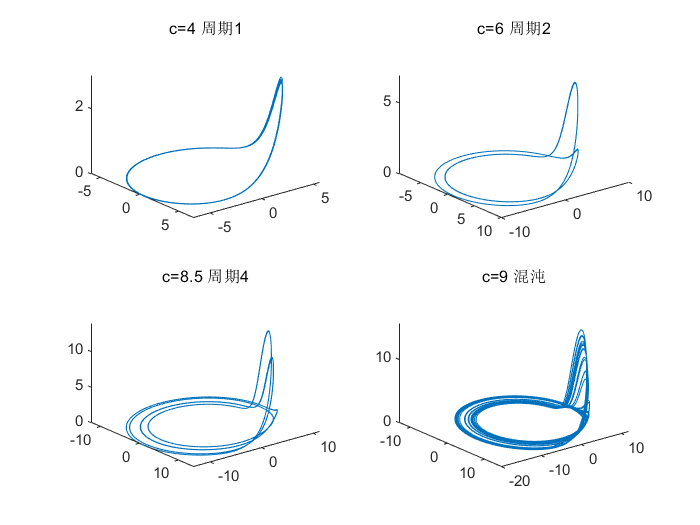这里根据其轨迹特性，选择x=0.1平面作为其庞加莱截面。

绘图代码如下，运行时间比较长，还需耐心等待。

%利用庞佳莱截面绘制分岔图
%截面采用公式Ax+By+Cz+D=0;的形式
%Rossler方程
clear
clc
close all
h=5e-3;%时间步长
x0=0:h:800;%时间
y0=[2;2;2];

%不同的c开始循环
c=3:0.02:16;
N_c=length(c);
N_P=300;%假设穿过截面的共有300个点
BF=nan(N_c,N_P);
for k=1:N_c
c_k=c(k);disp(c_k)
%计算出轨迹
[y1,~]=ODE_RK4_hyh(x0,h,y0,[0.1,0.1,c_k]);
%计算Poincare平面
Plane=[1;0;0;0.1];%x=0.1平面(正方向)
[tP_List,yP_List]=Solve_Poincare(x0,y1,Plane);%计算Poincare平面
%储存y值作为待会分岔图的点
N_P_temp=size(tP_List,2);
if N_P_temp>N_P
BF(k,1:N_P)=yP_List(2,1:N_P);
else
BF(k,1:N_P_temp)=yP_List(2,1:N_P_temp);
end
end

%绘制分岔图
figure()
hold on
for k=1:N_c
c_k=c(k);
plot(c_k*ones(1,N_P-30+1),BF(k,30:N_P),...
'LineStyle','none','Marker','.','MarkerFaceColor','k','MarkerEdgeColor','k',...
'MarkerSize',1)
end
hold off

%后置函数
function [tP_List,yP_List]=Solve_Poincare(t,y,Plane)
%截面方程z=0
% Plane=[0;0;1;0];%一般情况下是个垂直某个轴的平面
%一般只记录从负到正穿越。如果想反向也记录，可以设置Plane=-Plane。

%第二步，插值得到线与面的交点
yP_List=[];
tP_List=[];
Dis=DistancePlane(y,Plane);
N=size(y,2);
for k=1:N-1
if Dis(k)<=0 && Dis(k+1)>0
t0=t(k);t1=t(k+1);
yP0=y(:,k);yP1=y(:,k+1);
Dis0=Dis(k);Dis1=Dis(k+1);
%一维线性插值，求Dis=0时的t和y
%（相比较前面积分的4阶RK，这里用线性插值精度有点低）
yP=yP0+(yP1-yP0)/(Dis1-Dis0)*(0-Dis0);
tP=t0+(t1-t0)/(Dis1-Dis0)*(0-Dis0);
%储存
yP_List=[yP_List,yP];
tP_List=[tP_List,tP];
end
end
end

%点到平面的距离
function Dis=DistancePlane(xk,Plane)
% xk，坐标点，如果是3维坐标，大小就是3*N的矩阵。
% Plane，平面，形如Ax+By+Cz+D=0形式的平面。
N=size(xk,2);%计算总共多少个点
xk2=[xk;ones(1,N)];
Dis=dot(xk2,Plane*ones(1,N),1)./norm(Plane(1:end-1));
end

%两点线性插值
function y=interp2point_linear(x0,x1,y0,y1,x)
y=y0+(y1-y0)/(x1-x0)*(x-x0);
end

%两点3次插值
function y=interp2point_spline(x0,x1,y0,y1,x)
%y0包含y0的值和y0的导数,yy=y0(1),dy=y0(2)
xx0=x0;
xx1=x1;
yy0=y0(1);dy0=y0(2);
yy1=y1(1);dy1=y1(2);
cs = csape([xx0,xx1],[dy0,yy0,yy1,dy1],[1,1]);
y=ppval(cs,x);
end

function [F,Output]=Fdydx(x,y,Input)
%形式为Y'=F(x,Y)的方程，参见数值分析求解常系数微分方程相关知识
%高次用列向量表示，F=[dy(1);dy(2)];y(1)为函数，y(2)为函数导数
%Rossler 吸引子
a=Input(1);
b=Input(2);
c=Input(3);

dy(1)=-y(2)-y(3);
dy(2)=y(1)+a*y(2);
dy(3)=b+y(3)*(y(1)-c);

F=[dy(1);dy(2);dy(3)];
Output=[];
end

function [y,Output]=ODE_RK4_hyh(x,h,y0,Input)
%4阶RK方法
%h间隔为常数的算法
y=zeros(size(y0,1),size(x,2));
y(:,1)=y0;
for ii=1:length(x)-1
yn=y(:,ii);
xn=x(ii);
[K1,~]=Fdydx(xn    ,yn       ,Input);
[K2,~]=Fdydx(xn+h/2,yn+h/2*K1,Input);
[K3,~]=Fdydx(xn+h/2,yn+h/2*K2,Input);
[K4,~]=Fdydx(xn+h  ,yn+h*K3  ,Input);
y(:,ii+1)=yn+h/6*(K1+2*K2+2*K3+K4);
end
Output=[];
end


分岔图绘制如下：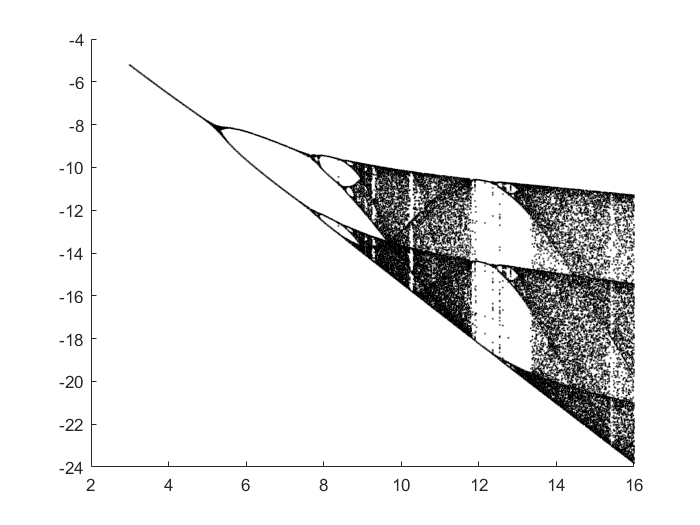庞加莱截面的选取会导致系统分岔图的形状发生改变。但是不会改变分岔点的位置。因为分岔点的位置是由系统本身所决定。

## 4.3 Lorenz系统分岔图

Lorenz系统是气象学家洛伦兹发现并提出的一个非线性系统，也是混沌学科的开端。在模拟大气流动时，洛伦兹发现初始的一个小小的误差，都会导致系统未来极大的变化。这种思想在20世纪60年代，给了那些物理学界中决定论者沉重的打击。洛伦兹也将这种不确定性，总结为“蝴蝶效应”。

这个系统可以被写为：
x ˙ = a ( y − x ) y ˙ = r x − y − x z z ˙ = x y − b z \dot{x}=a(y-x)\\ \dot{y}=rx-y-xz\\ \dot{z}=xy-bz
一般系统a=10，b=8/3，变化r值来观察系统的不同样子。下图分别展示了取不同r值所对应的xy平面的三维相轨线图：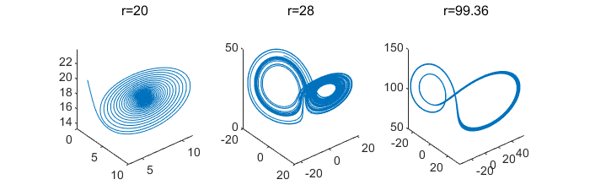分岔图为：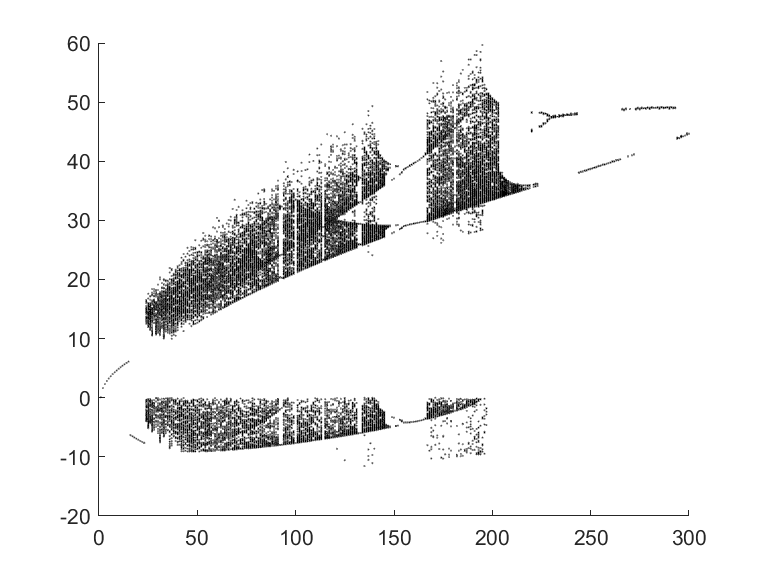绘图代码如下：

%利用庞佳莱截面绘制分岔图
%截面采用公式Ax+By+Cz+D=0;的形式
%Lorenz方程
clear
clc
close all

h=2e-3;
x1=100;
x0=0:h:x1;
y0=[2;2;2];
%不同的c
c=1:1:500;
N_c=length(c);
N_P=300;%假设穿过截面的共有300个点
BF=nan(N_c,N_P);
for k=1:N_c
c_k=c(k);disp(c_k)
%计算出轨迹
[y1,~]=ODE_RK4_hyh(0:10*h:x1,10*h,y0,[10,8/3,c_k]);%先粗略的计算前几步，然后排除初始点的影响，舍弃不要
[y1,~]=ODE_RK4_hyh(x0+x1,h,y1(:,end),[10,8/3,c_k]);
%计算Poincare平面
Plane=[1;-1;0;0];%x-y=0平面(正方向)
[tP_List,yP_List]=Solve_Poincare(x0,y1,Plane);%计算Poincare平面
%对于Lorenz系统再加一条，如果系统稳定了，则将稳定点也记录在最终分岔图内：
if isempty(tP_List) && sum(y1(1,end)-y1(2,end))<1e-2%如果最后x和y足够接近，则认为收敛了
tP_List=x0(end-1:end);
yP_List=y1(:,end-1:end);
end
%储存y值作为待会分岔图的点
N_P_temp=size(tP_List,2);
if N_P_temp>N_P
BF(k,1:N_P)=yP_List(2,1:N_P);
else
BF(k,1:N_P_temp)=yP_List(2,1:N_P_temp);
end
end

%绘制分岔图
figure()
hold on
for k=1:N_P
c_k=c(k);
plot(c_k*ones(1,N_P),BF(k,1:N_P),...
'LineStyle','none','Marker','.','MarkerFaceColor','k','MarkerEdgeColor','k',...
'MarkerSize',1)
end
hold off

function [tP_List,yP_List]=Solve_Poincare(t,y,Plane)
%截面方程z=0
% Plane=[0;0;1;0];%一般情况下是个垂直某个轴的平面
%一般只记录从负到正穿越。如果想反向也记录，可以设置Plane=-Plane。

%第二步，插值得到线与面的交点
yP_List=[];
tP_List=[];
Dis=DistancePlane(y,Plane);
N=size(y,2);
for k=1:N-1
if Dis(k)<=0 && Dis(k+1)>0
t0=t(k);t1=t(k+1);
yP0=y(:,k);yP1=y(:,k+1);
Dis0=Dis(k);Dis1=Dis(k+1);
%一维线性插值，求Dis=0时的t和y
%（相比较前面积分的4阶RK，这里用线性插值精度有点低）
yP=yP0+(yP1-yP0)/(Dis1-Dis0)*(0-Dis0);
tP=t0+(t1-t0)/(Dis1-Dis0)*(0-Dis0);
%储存
yP_List=[yP_List,yP];
tP_List=[tP_List,tP];
end
end
end

%点到平面的距离
function Dis=DistancePlane(xk,Plane)
% xk，坐标点，如果是3维坐标，大小就是3*N的矩阵。
% Plane，平面，形如Ax+By+Cz+D=0形式的平面。

N=size(xk,2);%计算总共多少个点
xk2=[xk;ones(1,N)];
Dis=dot(xk2,Plane*ones(1,N),1)./norm(Plane(1:end-1));
end

function [F,Output]=Fdydx(x,y,Input)
%形式为Y'=F(x,Y)的方程，参见数值分析求解常系数微分方程相关知识
%高次用列向量表示，F=[dy(1);dy(2)];y(1)为函数，y(2)为函数导数
%Lorenz 吸引子
a=Input(1);b=Input(2);r=Input(3);
dy(1)=a*(y(2)-y(1));
dy(2)=r*y(1)-y(2)-y(1)*y(3);
dy(3)=y(1)*y(2)-b*y(3);
F=[dy(1);dy(2);dy(3)];
Output=[];
end

function [y,Output]=ODE_RK4_hyh(x,h,y0,Input)
%4阶RK方法
%h间隔为常数的算法
y=zeros(size(y0,1),size(x,2));
y(:,1)=y0;
for ii=1:length(x)-1
yn=y(:,ii);
xn=x(ii);
[K1,~]=Fdydx(xn    ,yn       ,Input);
[K2,~]=Fdydx(xn+h/2,yn+h/2*K1,Input);
[K3,~]=Fdydx(xn+h/2,yn+h/2*K2,Input);
[K4,~]=Fdydx(xn+h  ,yn+h*K3  ,Input);
y(:,ii+1)=yn+h/6*(K1+2*K2+2*K3+K4);
end
Output=[];
end

展开全文matlab
• 建立了含间隙的7自由度螺旋锥齿轮动力学方程，结合分岔图和最大Lyapunov指数曲线研究了岔的演化过程及系统参数对系统分岔和混沌行为的影响。结果表明，分岔图与最大Lyapunov指数曲线从不同层面反映了系统随参数...
• 大数据-算法-非线性电力系统分岔控制的研究.pdf
• 应用中心流形对一类含有二次和三次非线性项的Duffing系统降维，并数值模拟出其分岔图及Lyapunov指数图，对其进行分析，进一步研究其稳定性及岔特性．
• 大数据-算法-三维和四维非线性系统Hopf分岔反.pdf
• 针对高维Hopf动态分岔问题，研究了不经计算其传统规范形，直接计算高维任意阶数的Hopf分岔系统的最简规范形。利用中心流形定理，将原n维动力系统降为二维的中心流形，根据规范形理论，对中心流形上流的方程进一步...
• 混沌Lorenz系统的matalb仿真，采用getmax、映射、最大值三种算法，绘出Lorenz系统分岔图，分析系统的混沌时刻。
• 应用MLP法求解谐波激励下强非线性系统的解析近似解，并运用MLP法与多尺度法结合的方法得到该系统的分岔响应方程。采用奇异性理论研究系统在非自治情形下的分岔特性，得到不同参数下系统的分岔形态。最后通过具体算例...
• 0_非线性振动系统的分叉和混沌理论_陈予恕_1993_kkkyyy
• 利用Poincar6映射原理，提出了求高维非线性系统周期解及其分岔的方法。将从初值至稳态解的整个积分长度分成若干积分子段，设定每个积分子段中的最大循环数，并使周期数按一定规律增加。在每个子段中应用直接积分法...
• 耦合发电机系统分岔图和李雅普诺夫指数图的做法 可以把系统方程更改 然后得到自己想要的微分方程的分岔图和李雅普诺夫指数图图谱 之外还有相空间图
• 大数据-算法-非线性振动系统分岔混沌及相关控制.pdf
• 以及轧机主传动系统具有复杂机电耦联的特点，应用Lagrange-Maxwen原理建立一类含非线性摩阻的轧机主传动机电耦联扭转系统非线性动力学方程，利用非线性动态分岔理论，分析该系统的稳定性，给出系统发生Hopf分岔的...
• 新能源电力系统含限幅或控制切换等非线性环节，本质上为非光滑系统，其稳定性和失稳形式复杂。对此，研究了大扰动下双馈风机并网系统中由限幅饱和导致的混沌振荡失稳机理，在数学上对应着非光滑系统轨迹发生从收敛至...
• 研究了一类非线性金融系统。首先利用特征方程和Routh Hurwitz准则对系统的平衡点的稳定性和hopf分岔的存在性进行了研究;其次利用解析法研究了系统hopf的分岔方向和分岔稳定性;最后证明了在一定条件下,系统的hopf分岔...
• 然后借助于动力学稳定性理论, 研究了迁移对系统的每个平衡态的局部动力学稳定性的影响, 得出两地间的迁移在四维竞争Lotka-Volterra 系统中可以引起的Turing 分岔的结论, 并给出该系统在共存平衡点附近经历Turing ...
• 研究了一类电磁轴承非线性系统模型的多极限环分岔问题，该模型平均方程为5阶Z2-等变扰动平面Hamilton向量场，利用平面动力系统分岔理论，借助于Maple符号软件计算程序，通过控制其参数，发现系统在2组精确的参数控制...
• 动力系统理论没有被采用的最大原因之一在工程环境中广泛应用，主要是由于缺乏分叉软件它可以相对轻松地与现有工具集集成。 因此我们试图解决这个问题通过将 AUTO 合并到 MATLAB 中来解决问题，从而构建了 Dynamical...matlab
• 改进了传统规范形理论，使其适用于研究两自由度强非线性振动系统的渐近响应并进行了相应的分岔分析。通过将待定固有频率法引入规范形求解过程，获得了两自由度立方Duffing-VanderPol强非线性振动子的规范形及稳态...
• 大数据-算法-非线性动力系统的时滞反馈分岔控制研究.pdf...